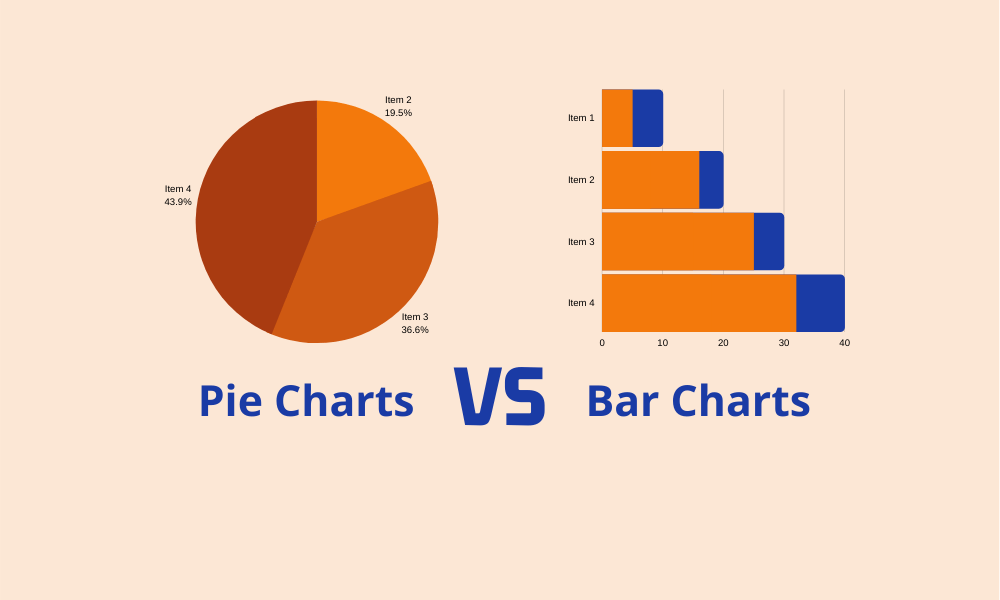## Introduction

The argument of pie charts vs. bar charts is almost 100 years old, going back to Walter Eells’ paper titled “The Relative Merits of Circles and Bars for Representing Component Parts.” While pie charts are more common in business presentations, bar charts are finding increased use. There are advantages and disadvantages to both. In this post, you will learn about those as well as see alternatives.

## Pie Charts

### When to use a pie chart?

Analysts create pie charts to show the distribution of proportions, such as the percent of votes different candidates get in an election, or the proportion of revenue streams for a company. This is the simplest way to show “parts of the whole.”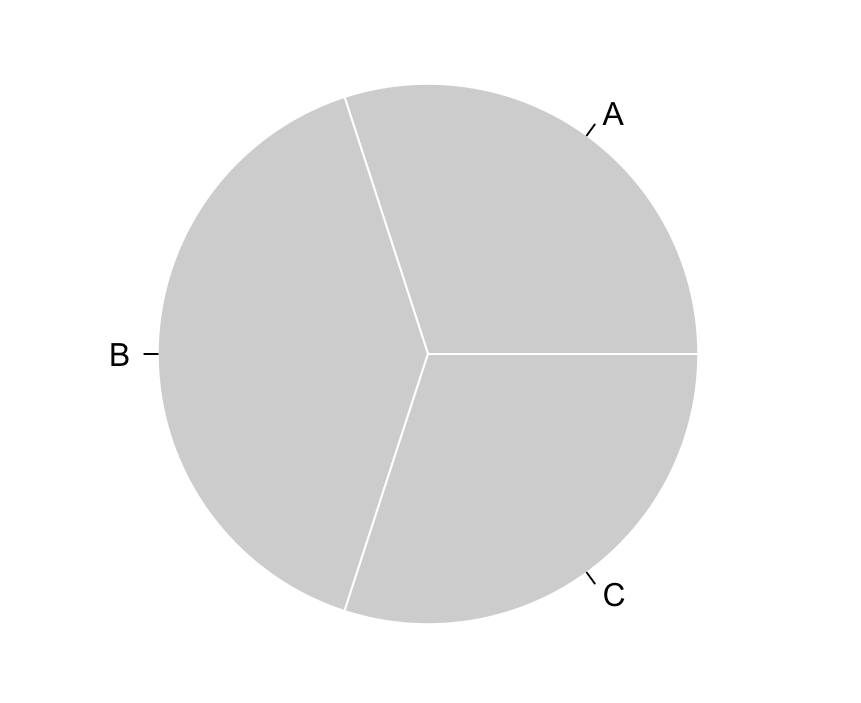Figure 1: Simple pie chart

Pie charts become confusing or unclear when many proportions, hence slices, are present. They are especially challenging when the slices are small. To solve this problem, creators use colors and legends to differentiate slices, but end up worsening the problem.

For example, compare charts I, II, and III below.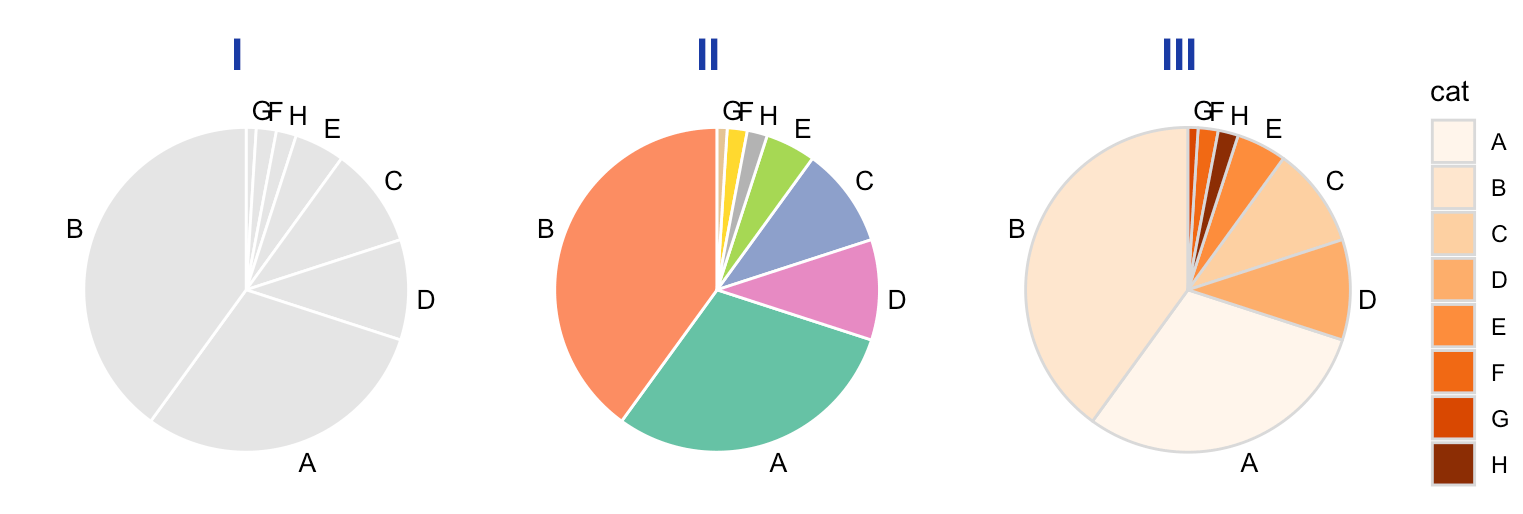Figure 2: Three different pie charts with colors and legends

In the chart I, it is hard to differentiate between G and F. Chart II solves the differentiation problem, but we don’t know the value of G or F. Chart III tries to help with a legend, which may be needed if the labels are long, but cause another problem: looking for the key every time.

A potential solution is adding direct labels with data to each slice. While labels help clarify and offer precision, they defeat the purpose of creating a chart.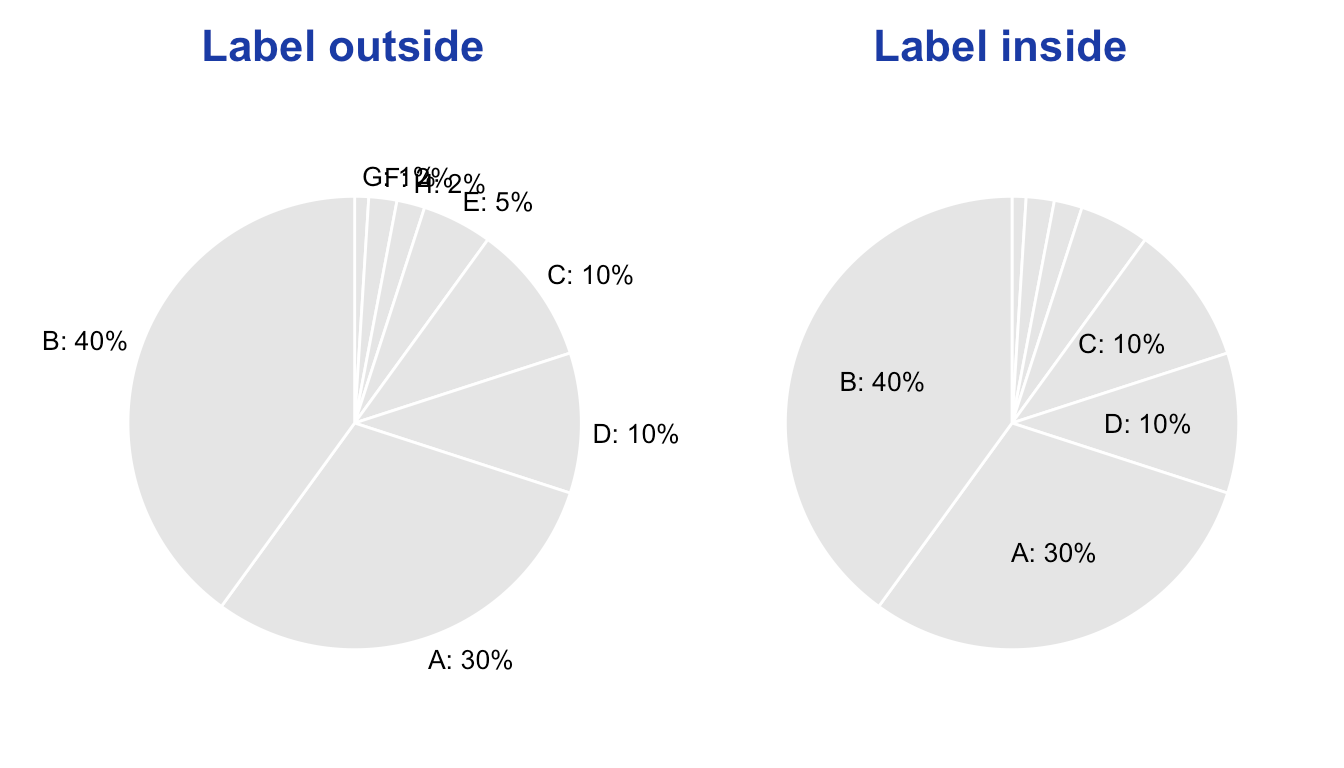Figure 3: Pie charts with data labels

We can create better pie charts by reducing the number of slices by collapsing some of them or highlighting the important ones.

Let’s see these one at a time.

### Reduced number of slices

We will put all the small ones in the “other” category.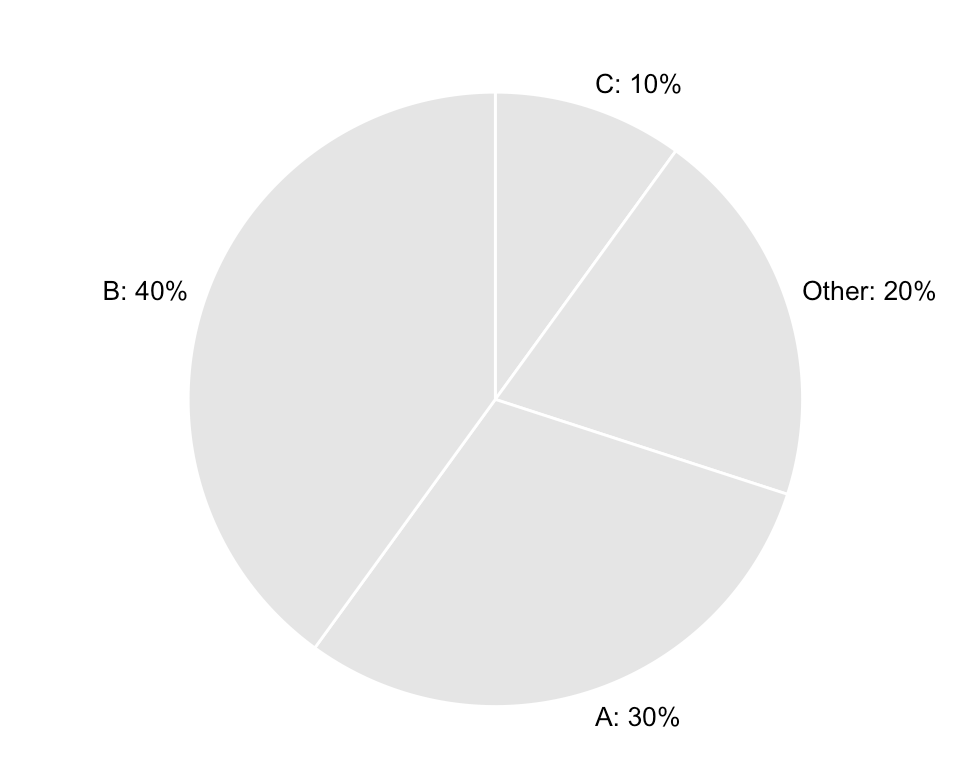Figure 4: Pie chart with collapsed categories

### Highlight an important slice

We will keep the color of all slices the same, except for the one we want to highlight. Highlighting can be used to make a point along with an annotation.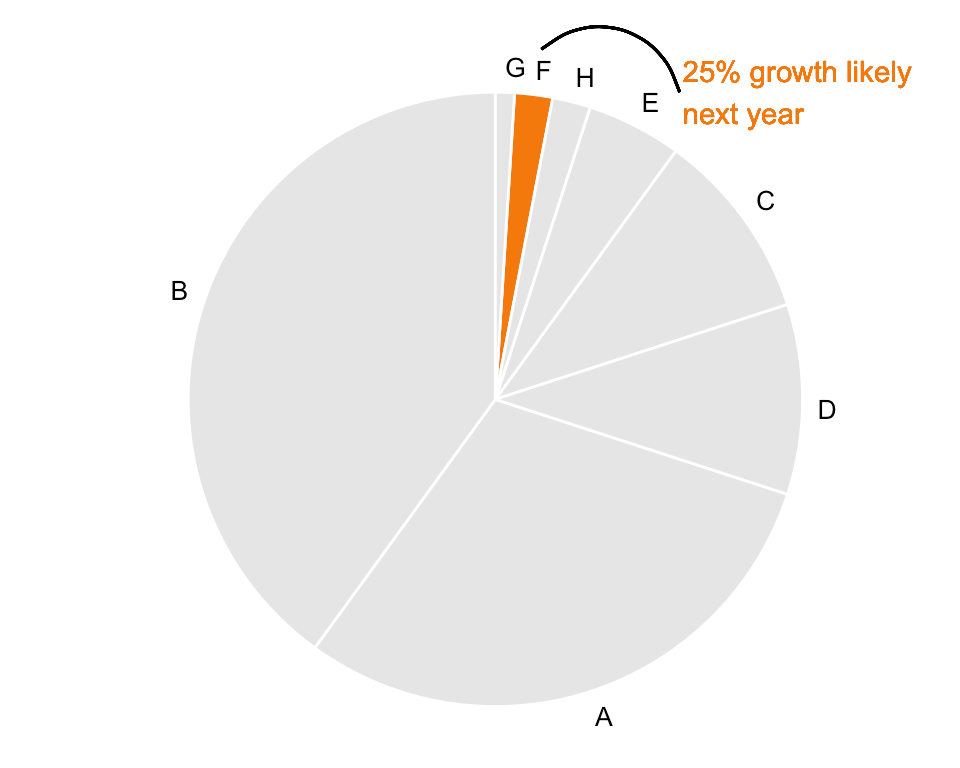Figure 5: Pie chart with a slice highlighted

### Advantages of a pie chart

• You can see the portion of a category as compared to other the portions
• It offers an easy way to compare large and small slices
• It is intuitive

### Disadvantages of a pie chart

• It makes comparison harder of almost similar sized slices
• With too many proportions or slices, it is harder to see the difference
• When colors or legends are used to differentiate slices, understanding is harder
• Too many labels crowd the chart, and the main point is lost

## Bar charts

### When to use bar charts?

Analysts create bar charts for a variety of uses: to show the absolute or proportional value i.e. total sales by product or proportion of a product sales of the total sales. Sometimes analysts create bar charts to show year over year numbers.

Although bar charts don’t show a part of the whole intuitively, they make comparisons of each proportion easy.

See the example below. You can see that the categories C and D are of the same size, and category G is the smallest.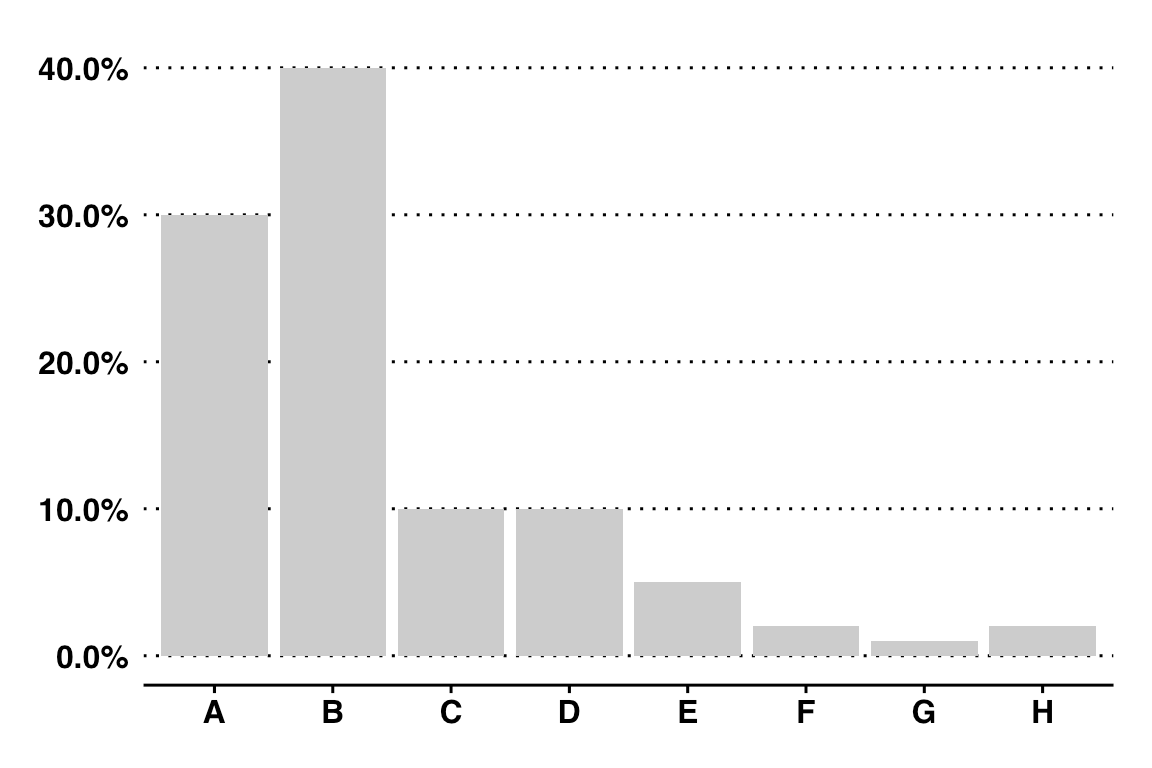Figure 6: Simple bar chart

This chart can be made better by arranging (or sorting) the bars by their lengths.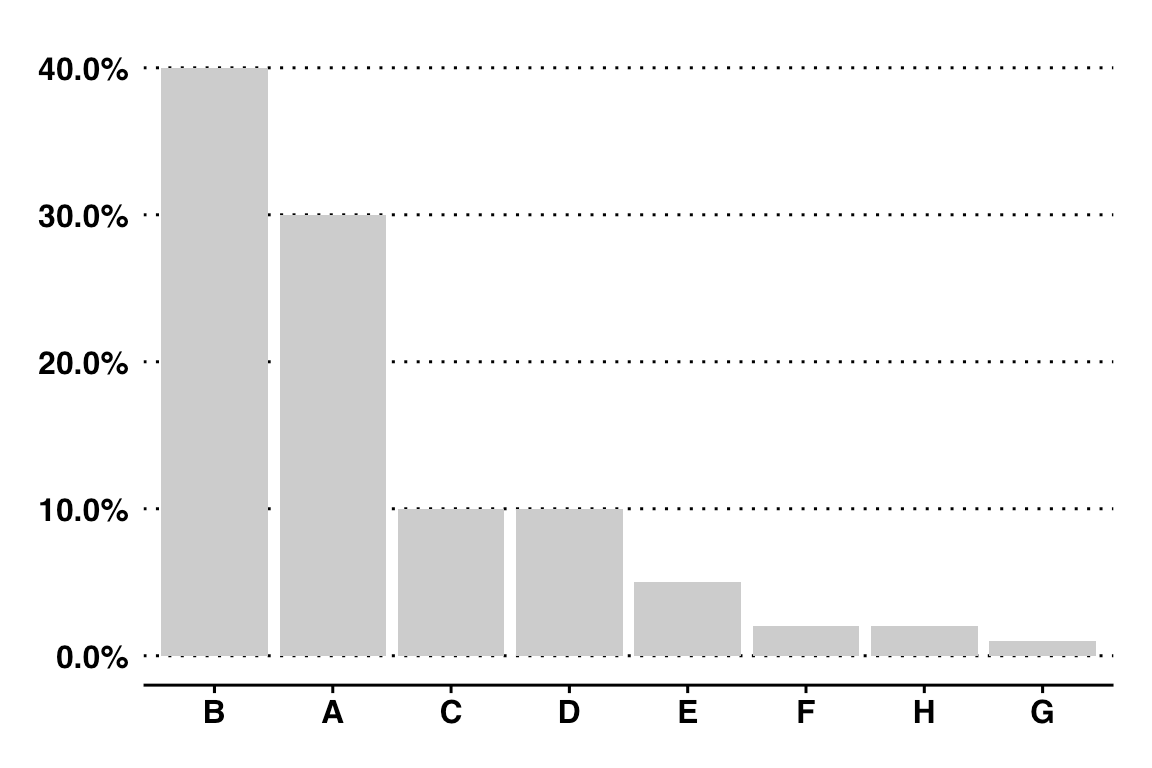Figure 7: Ordered bar chart

We can make this chart aesthetically pleasing by placing invisible or white gridlines.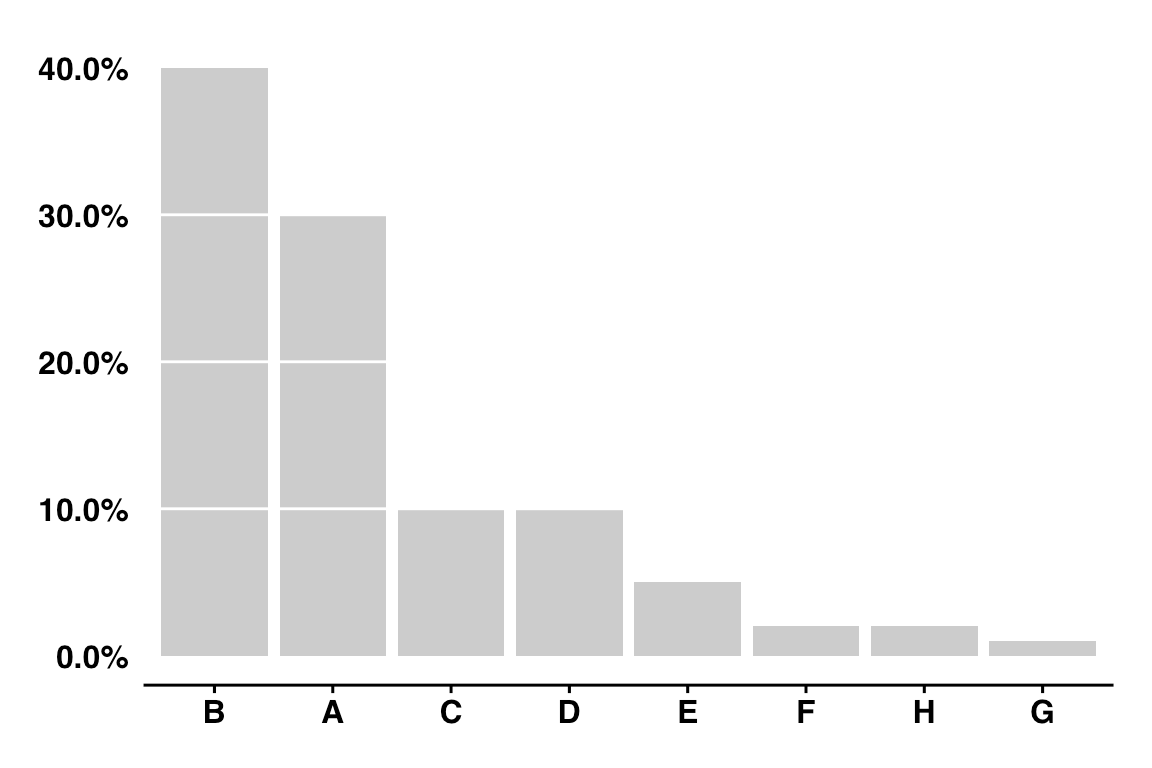Figure 8: Ordered bar chart with white gridlines

Bar charts (the vertical ones) also have problems when the labels are long. Often they are at an angle, and you need to turn your head to read them.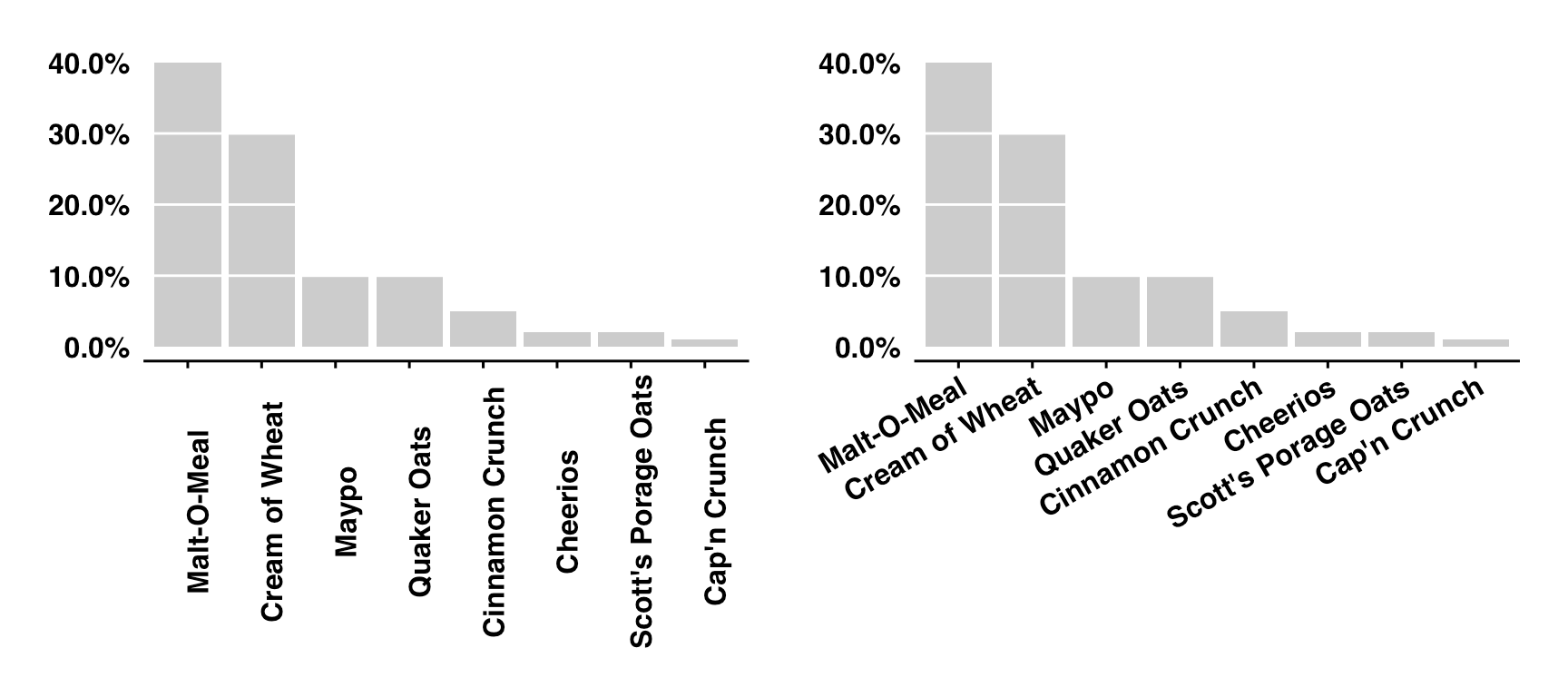Figure 9: Bar charts with long labels

You can solve this problem by moving the labels to the y-axis: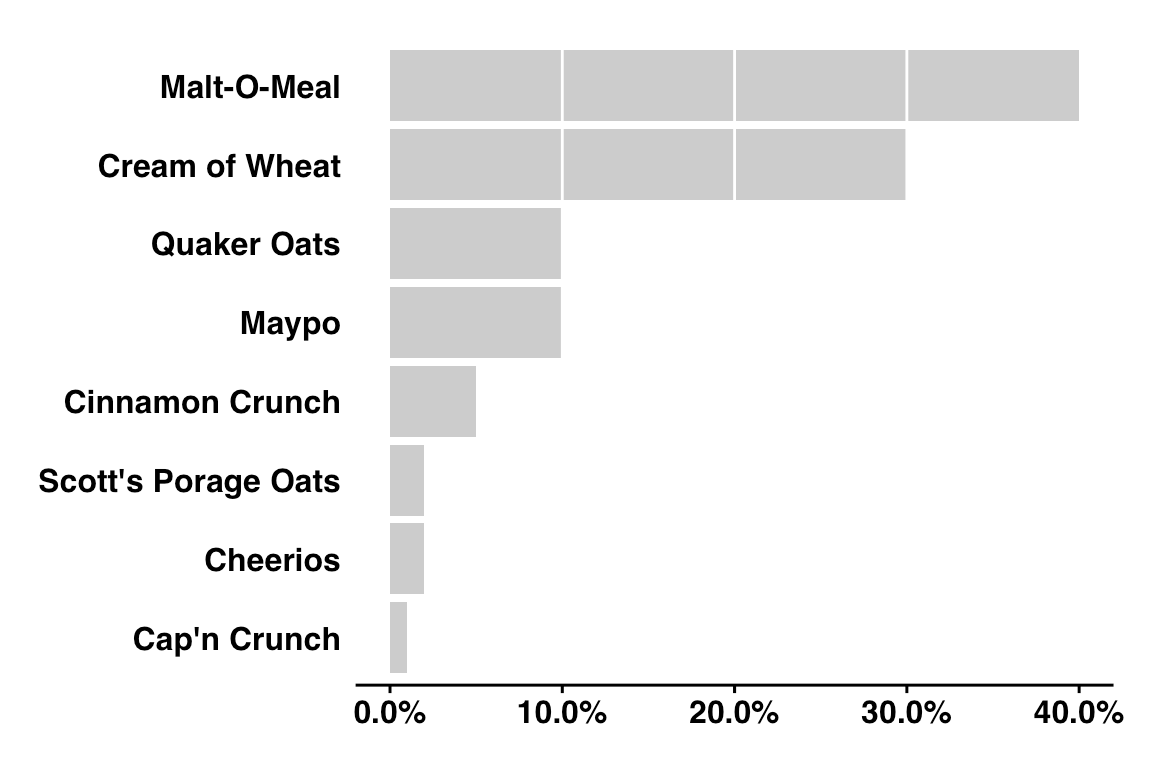Figure 10: Bar charts with long labels flipped on the y-axis

Although the bar charts make the comparison easier than a pie chart, knowing the size of the smaller bars is similarly hard as knowing the size of a small slice from a pie chart. But at least the bar charts have gridlines to help us.

Just like the pie charts, we can try to overcome this problem by adding labels to the bars.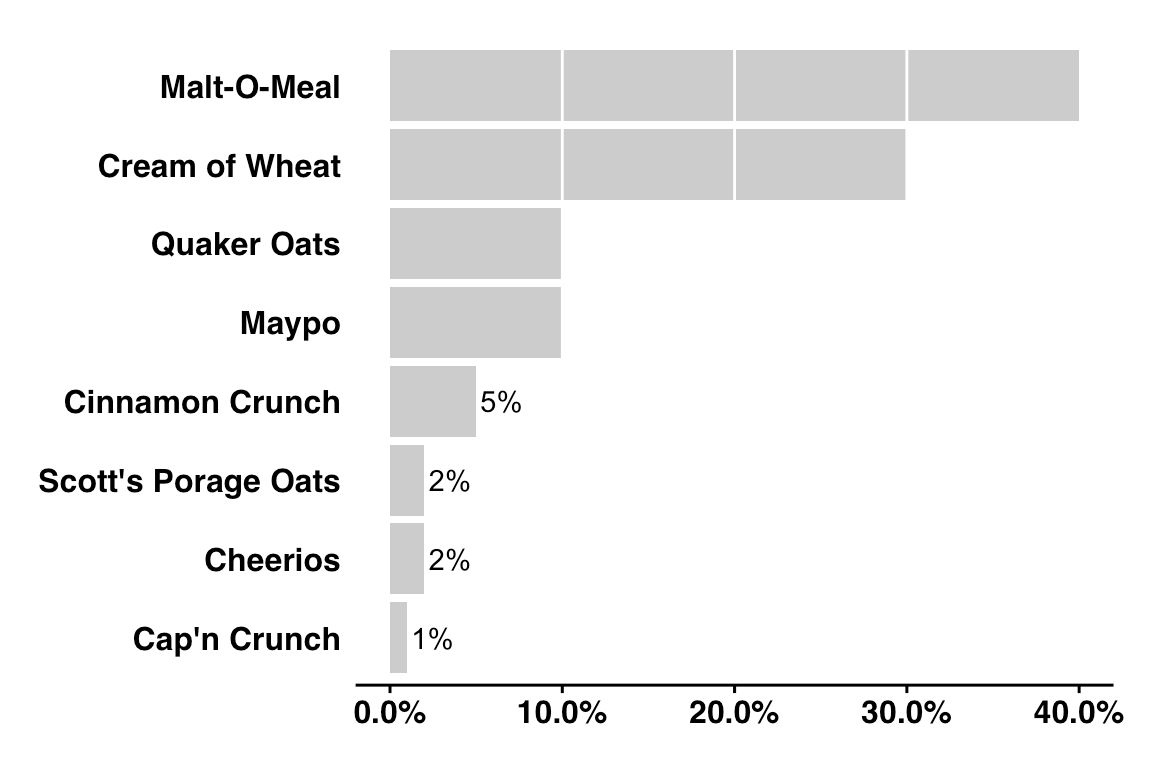Figure 11: Bar charts with long labels flipped on the y-axis with some data labels

• Comparison among categories is easier
• Gridlines help guide the reader
• Length of the bar makes understanding easier
• Color coding and legends are not required (for single bars)
• Direct labeling is easier

• Smaller bars don’t offer precision
• Hard to “see” the proportion, that is the part of the whole

## When to use a pie chart vs. bar chart

Use pie charts when:

• The number of categories is small
• Readers can differentiate slices (unless you are making a point)
• You don’t need to rely on many colors or labels to explain the proportions
• The total adds up to 100%

Use bar charts when:

• You have many categories (not too many)
• You need to compare numbers side-by-side (caution: more than two bars are hard for readers

## Waffle Charts

Waffle charts combine bar charts and pie charts into one chart and compound their problems. While you can see the proportions, precision is lost, especially when the proportions don’t fit in one square. And, you still have to decode the color and legend to understand the chart. Also, longer labels will create problems with legend positioning. These charts could be useful with fewer categories, or if analysts want to make a point.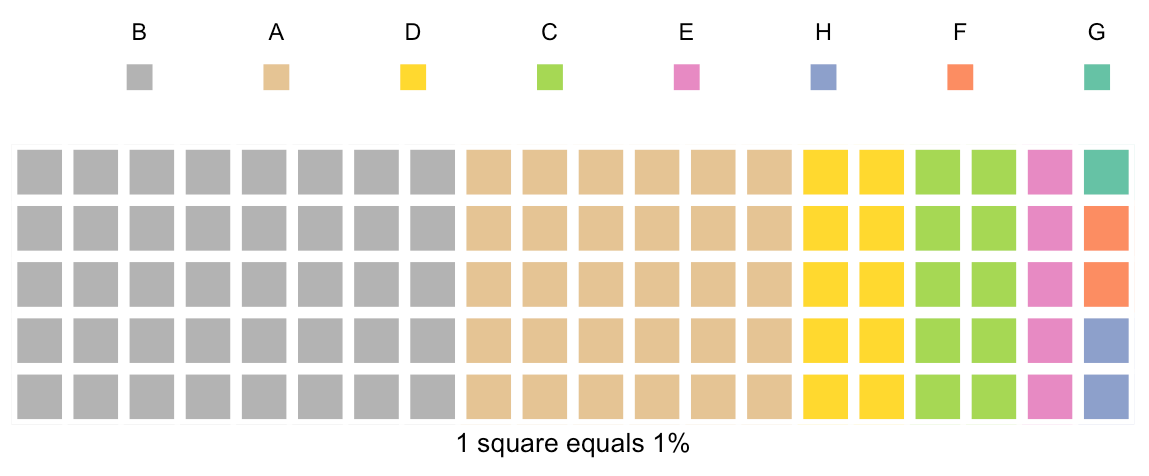Figure 12: A waffle chart

## Dot charts

Dot charts or dot plots, popularized by William Cleveland, are similar to bar plots, except the bars are replaced with a dot at the end of the bar.

It’s easier to see an example: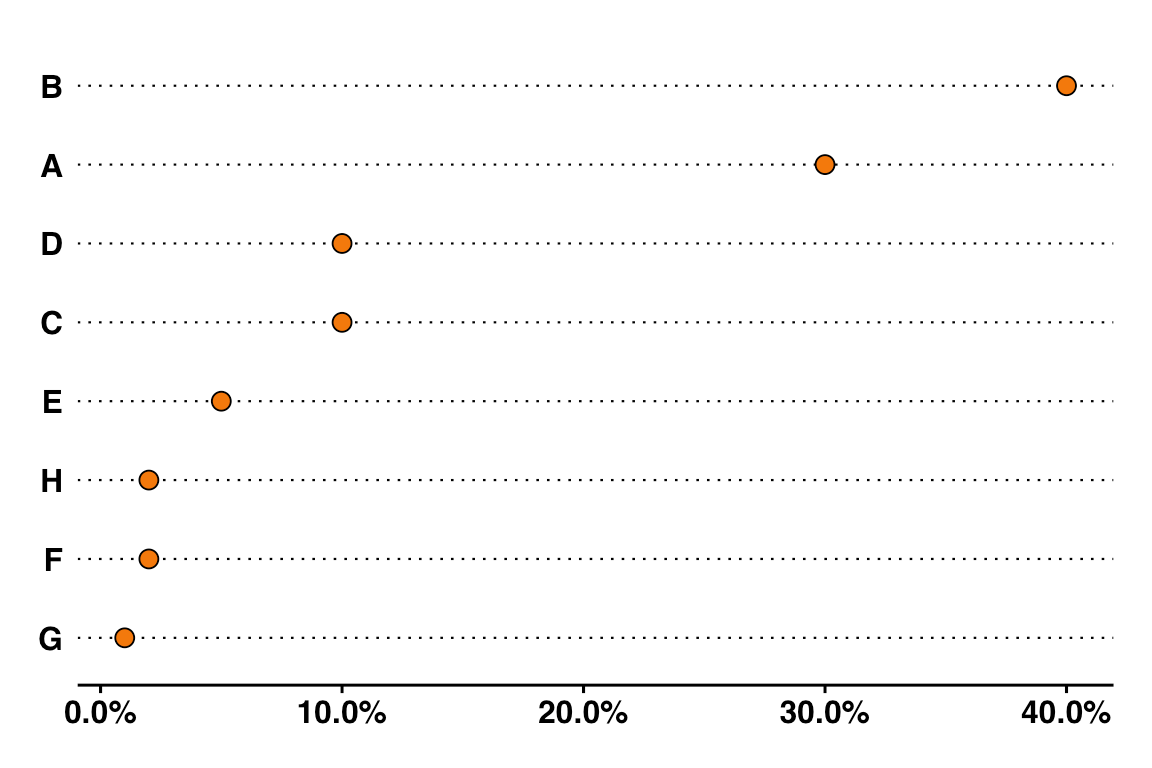Figure 13: A simple dot chart

Dot charts use the best qualities of a bar chart such as ordered data, labels on the y-axis, and reducing the need to rely on color for decoding the data. It exceeds the usefulness of a bar chart by using a smaller space than a bar chart.

Cleveland said this about area and perception, “Using area to encode quantitative information is a poor graphical method. Effects that can be readily perceived in other visualizations are often lost in an encoding by area.”

• Comparison is easier
• Take little space
• Differences among categories are noticeable
• Color or legend coding not required (for single categories)

• Some precision is lost
• They might be new to viewers who may find the charts dull

Let’s look at how pie charts compare against bar charts with real data. These data are from: “Reveal from The Center for Investigative Reporting and The Center for Employment Equity,” a study on diversity in silicon valley.

First up, let’s look at all the distribution of IT professionals by roles.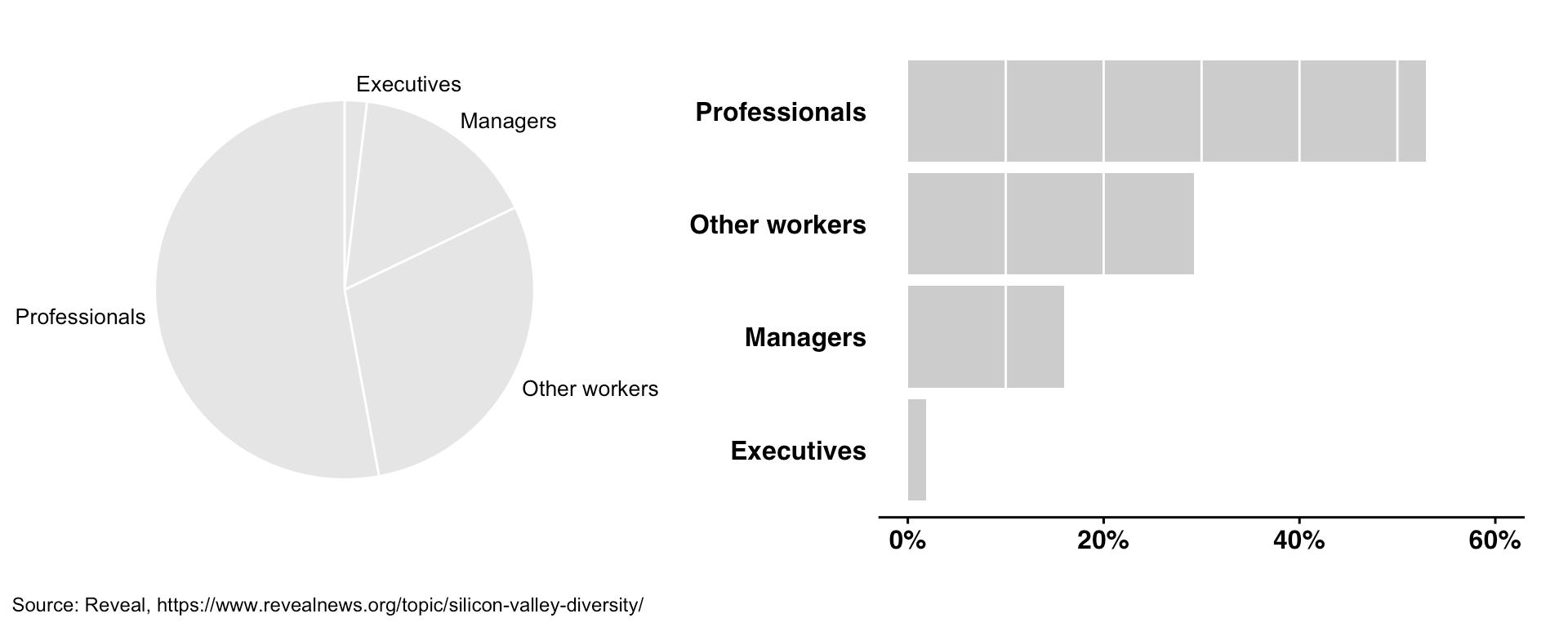Figure 14: Bar chart vs. pie chart comparing job categories of employees in Silicon Valley companies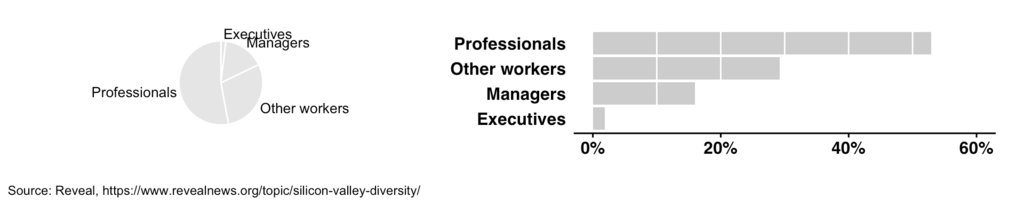Figure 15: Bar chart vs. pie chart comparing job categories of employees in Silicon Valley companies with reduced height

### What do we see

• Both the pie and bar chart in Figure 14 clearly show that professionals account for more than 50% of the workforce.
• It is hard to see the percentage of executives.
• Both the charts clearly show that there more “other workers” than there are managers.
• It is hard to see the percentage of “other workers” in the pie chart, but you can see that it is slightly below 30% in the bar chart.
• When we reduced the height of both plots by 50% in Figure 15, you can see that the bar chart is still readable and gives you the same information as Figure 14

Verdict: slight advantage bar charts. If we add data labels to both the charts, they will be at the same level.

Now, let’s look at the distribution of ethnicities/races.

Here’s another head to head: pie charts vs. bar charts round II.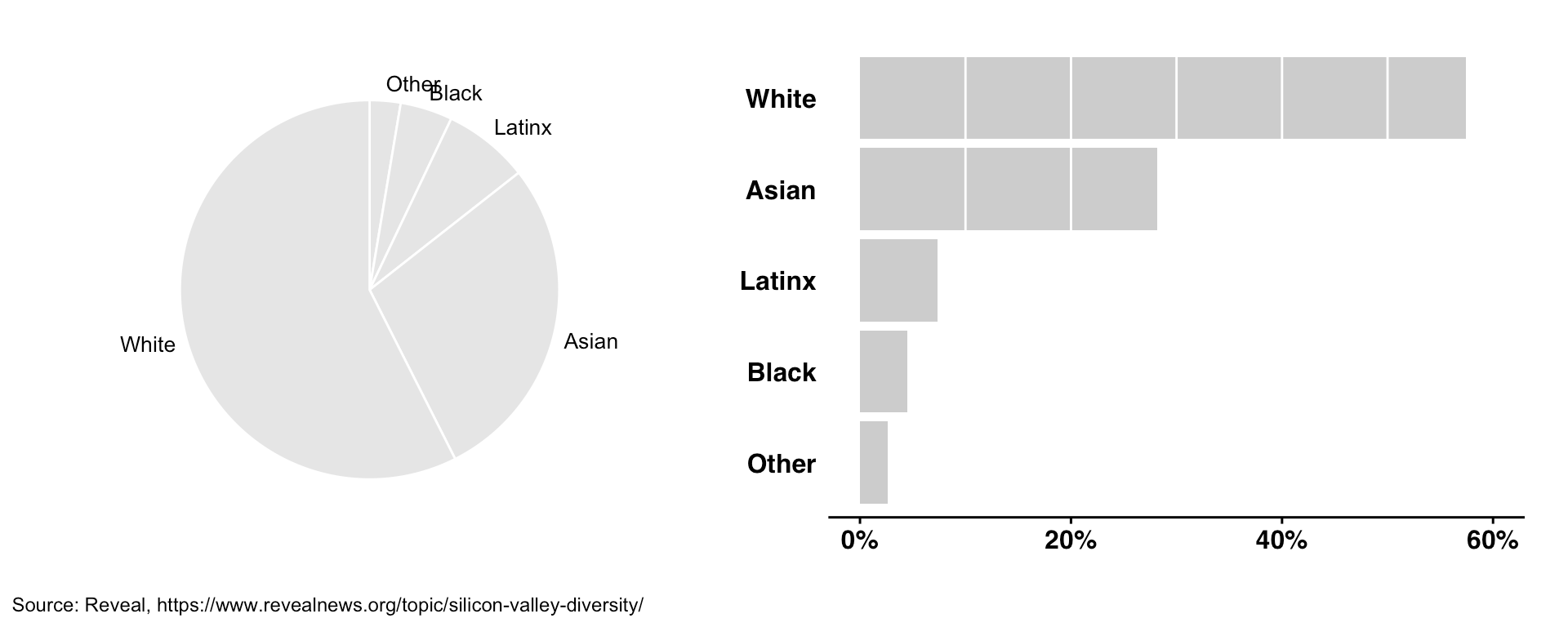Figure 15: Bar chart vs. pie chart comparing ethnicities/races of employees in Silicon Valley companies

### What do we see

• Both the pie and bar chart in Figure 15 clearly show that white employees account for more than 50% of the workforce.
• Both the charts show that Asian employees account for slightly over 25% of the workforce
• The bar chart shows that there are almost double the number of Latinx employees than Black employees. We can see the same ratio for Asian and white employees in the bar chart.
• It is hard to see the percentage of “other” and Black employees.

Verdict: slight advantage bar charts. With data labels, the pie chart may come out ahead.

What if we want to see the job category and race/ethnicity at the same time. We can use stack bar charts or side-by-side bar charts, but what about the pie charts?

We can combine the ethnicity/race with the job category and create more slices. We can also use hues for different ethnicity/races and place them close to each other. Round III of pie charts vs. bar charts .

Job category and race/ethnicity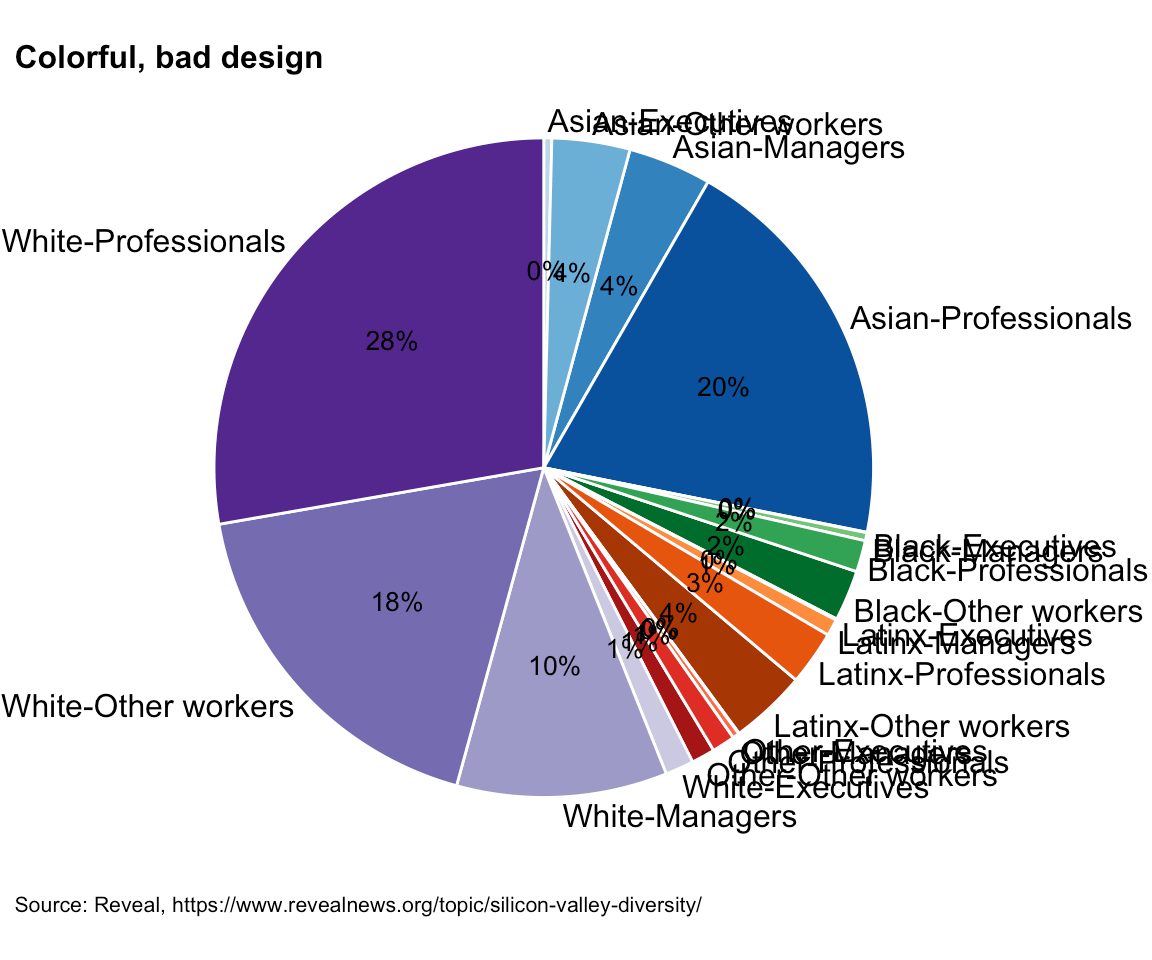Figure 16: A pie chart showing ethnicities/races and job categories of employees in Silicon Valley companies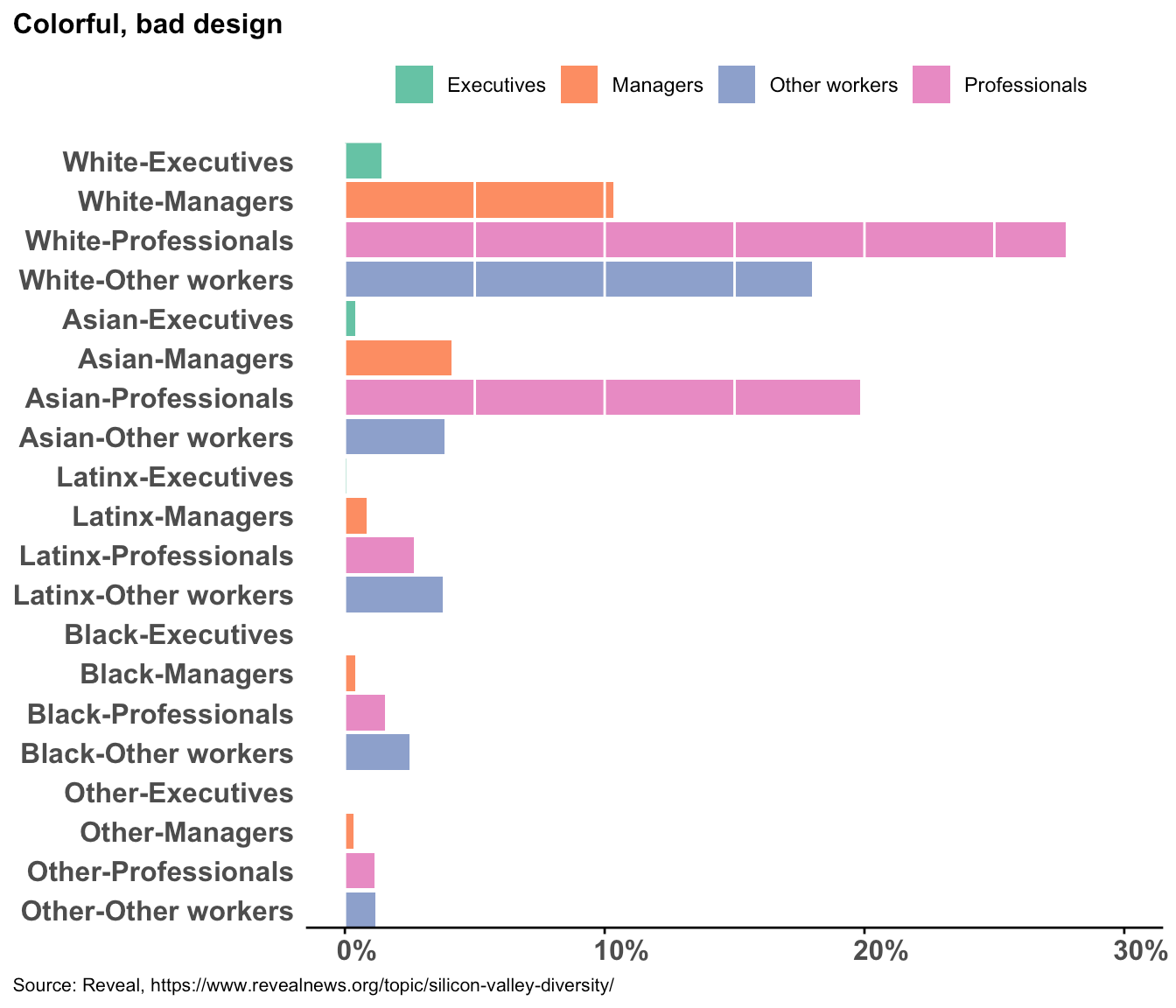Figure 17: A bar chart showing ethnicities/races and job categories of employees in Silicon Valley companies

### What do we see

• Many labels in the pie chart (Figure 16) are unreadable
• We see white “professionals” account for most of the IT workforce, followed by Asian “professionals”. This can be seen clearly in both the charts (Figure 16 and 17)
• In the bar chart (Figure 17), you can see managers who are white makeup for the most number of managers.
• In the bar chart, you can see the non-existence of Latinx, Black, and other executives though the data does contain a small percentage of executives from these groups.
• Although you can’t say it with precision, in the bar chart, you can see the ratio of Asian managers to Asian professionals compared to white managers to white professionals is smaller. [It is 0.2 compared to .37]

Verdict: meh! Except for one or two large patterns, you have to search into the charts to make meaningful observations.

We can try facet or “small multiples” to see whether these charts are any better. Let’s see panels of ethnicity/race and job category distribution. Round IV of panel pie charts vs. bar charts.

## Small multiples

### Panels of ethnicity/race with job category distribution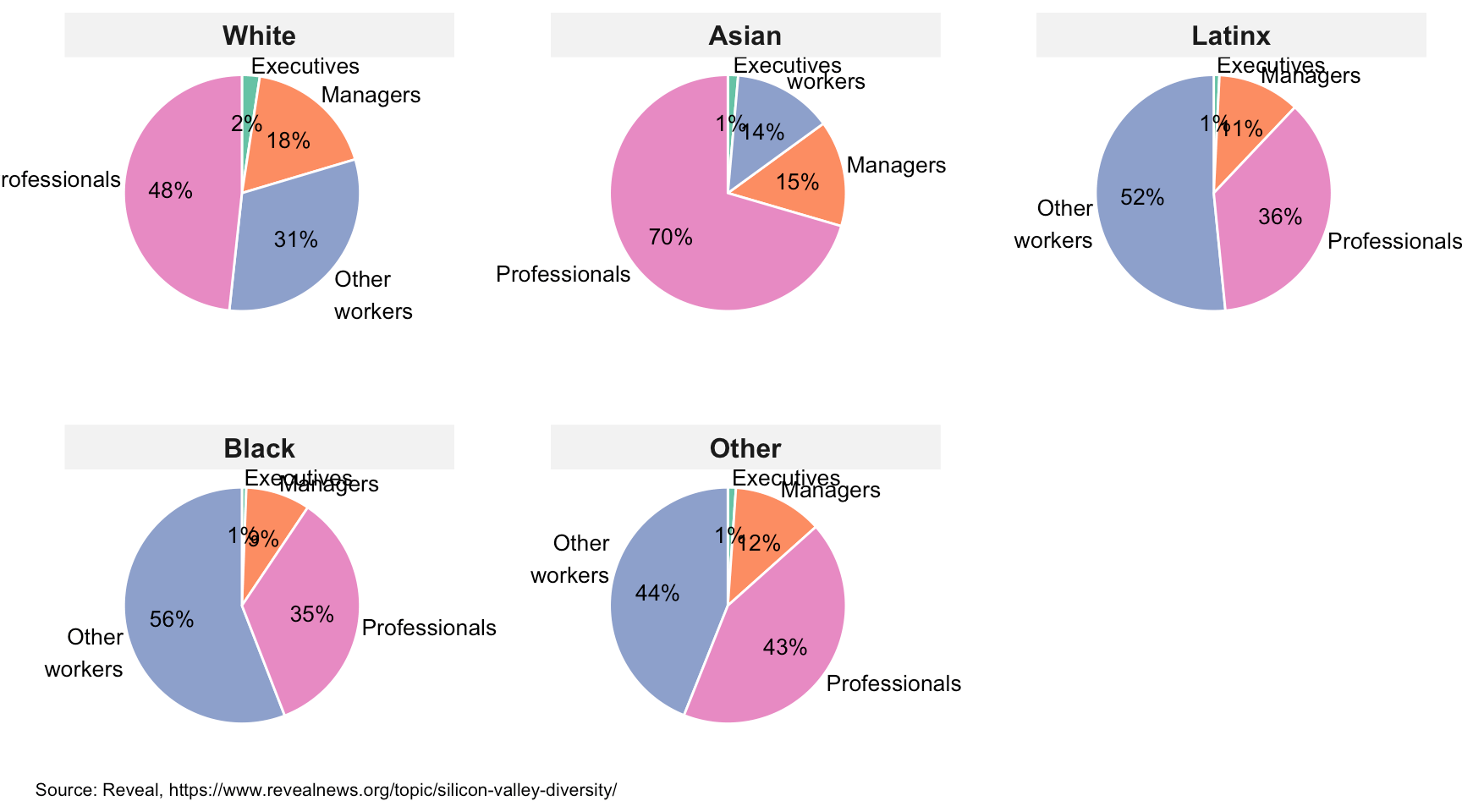Figure 18: Pie chart panels showing job categories of employees in Silicon Valley companies by ethnicity/race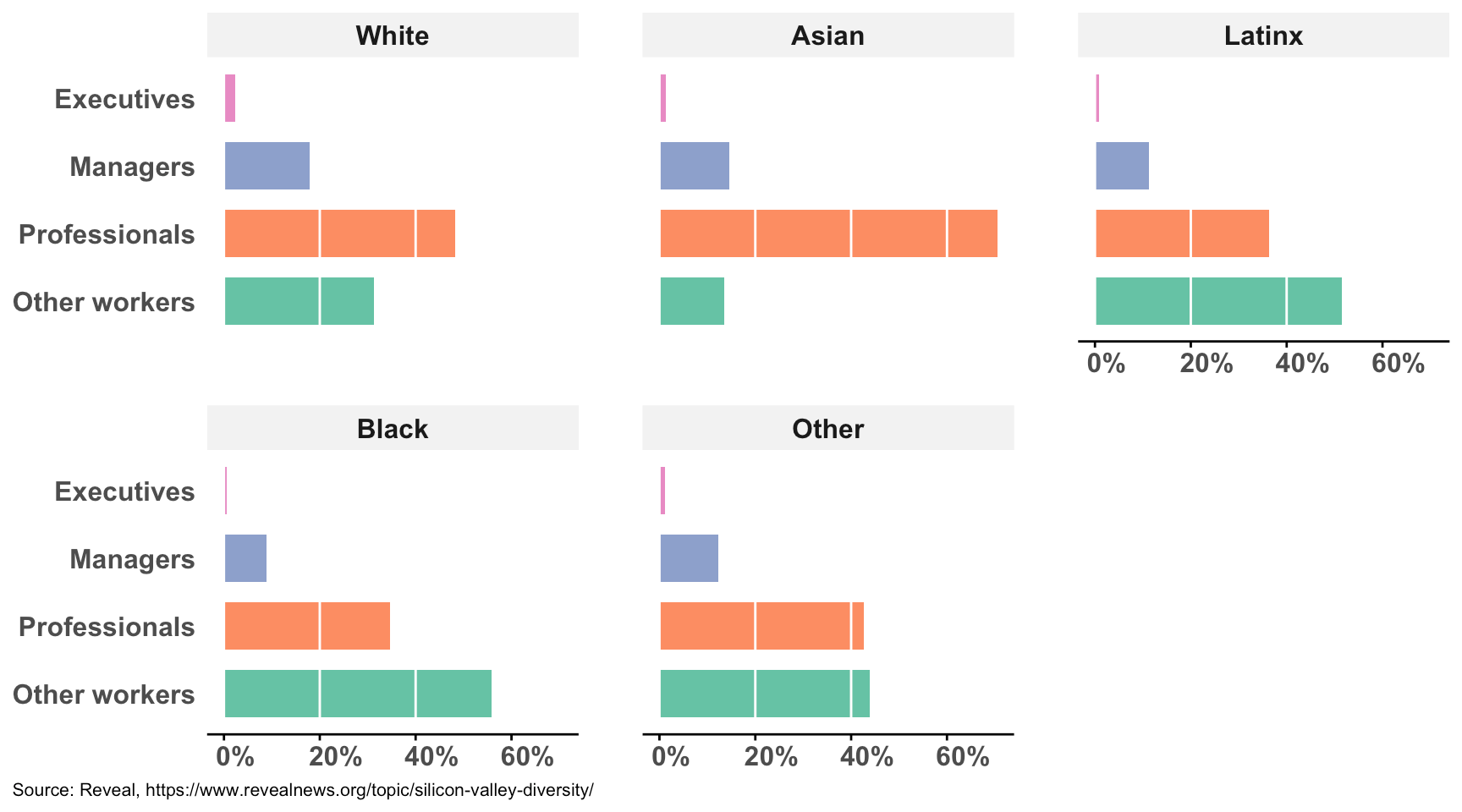Figure 19: Bar chart panels showing job categories of employees in Silicon Valley companies by ethnicity/race

### What do we see

• You have to jump from one chart to another to make comparisons, more so with pie charts
• In the pie charts (Figure 18), you see:
• A large percentage of Asian employees are “professionals”
• More than half of the Black and Latinx employees fall in the “Other workers” category
• In the bar charts (Figure 19), you see:
• The ratio of managers to “professionals” look smaller compared to the other ethnicities/races
• The ratio of “other workers” to “professionals” look bigger for Black and Latinx employees compared to the other ethnicities/races

Verdict: tie. Both charts offer a different understanding. The pie charts show underrepresentation or over-representation more intuitively, but the comparison between graphs is harder than the bar charts.

### Panels of job category by ethnicity/race distribution

Let’s see panels of job category by ethnicity/race distribution. Round V of pie charts vs. bar charts.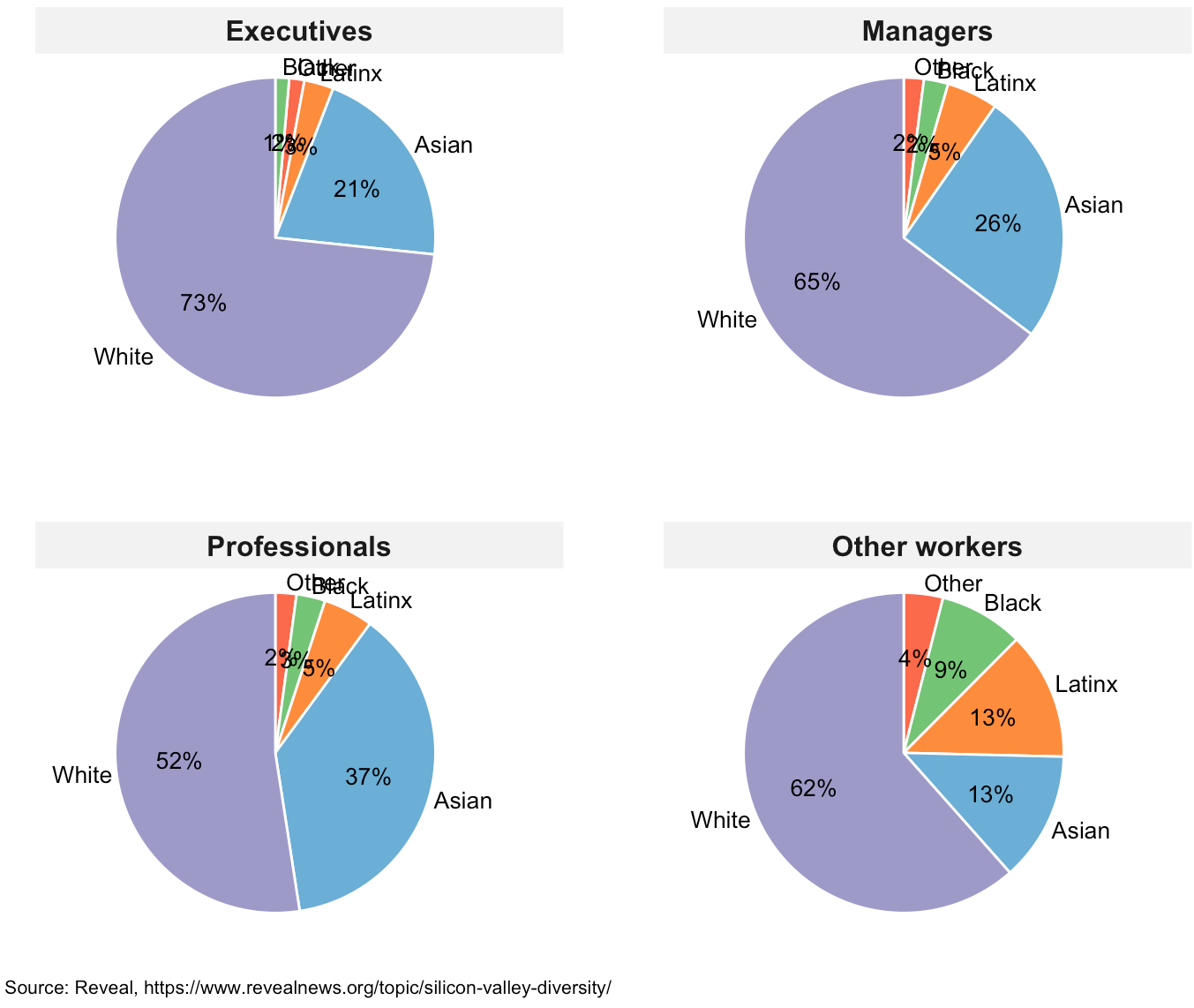Figure 20: Pie chart panels showing ethnicity/race of employees in Silicon Valley companies by job category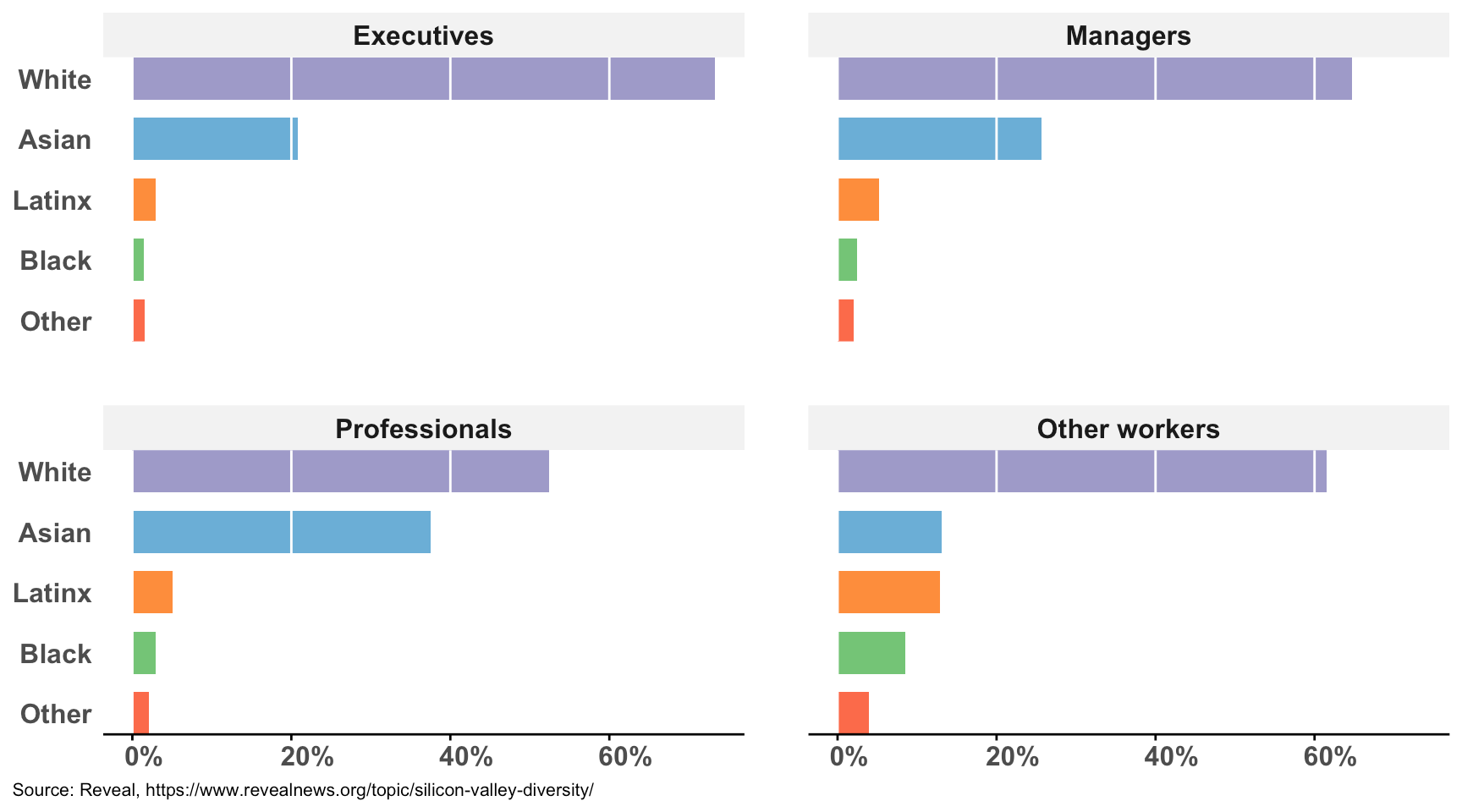Figure 21: Bar chart panels showing ethnicity/race of employees in Silicon Valley companies by job category

### What do we see

• In the pie charts (Figure 20), you can see:
• white employees account for a large portion of the executives
• Asian employees have a large percentage of “professionals”
• Latinx employees have a higher representation in the “other workers” categories compared to the other job categories.
• In the bar charts (Figure 21), you can see:
• Black employees are underrepresented in all of the categories, except for the “other workers” category.

Verdict: pie charts are slightly ahead. Maybe because the 73% and 52% of white employees in the executive and “professionals” categories respectively jumped out to me. The data labels are harder to see for smaller slices, and if we added those to the bar charts, it could be a tie.

## Dot charts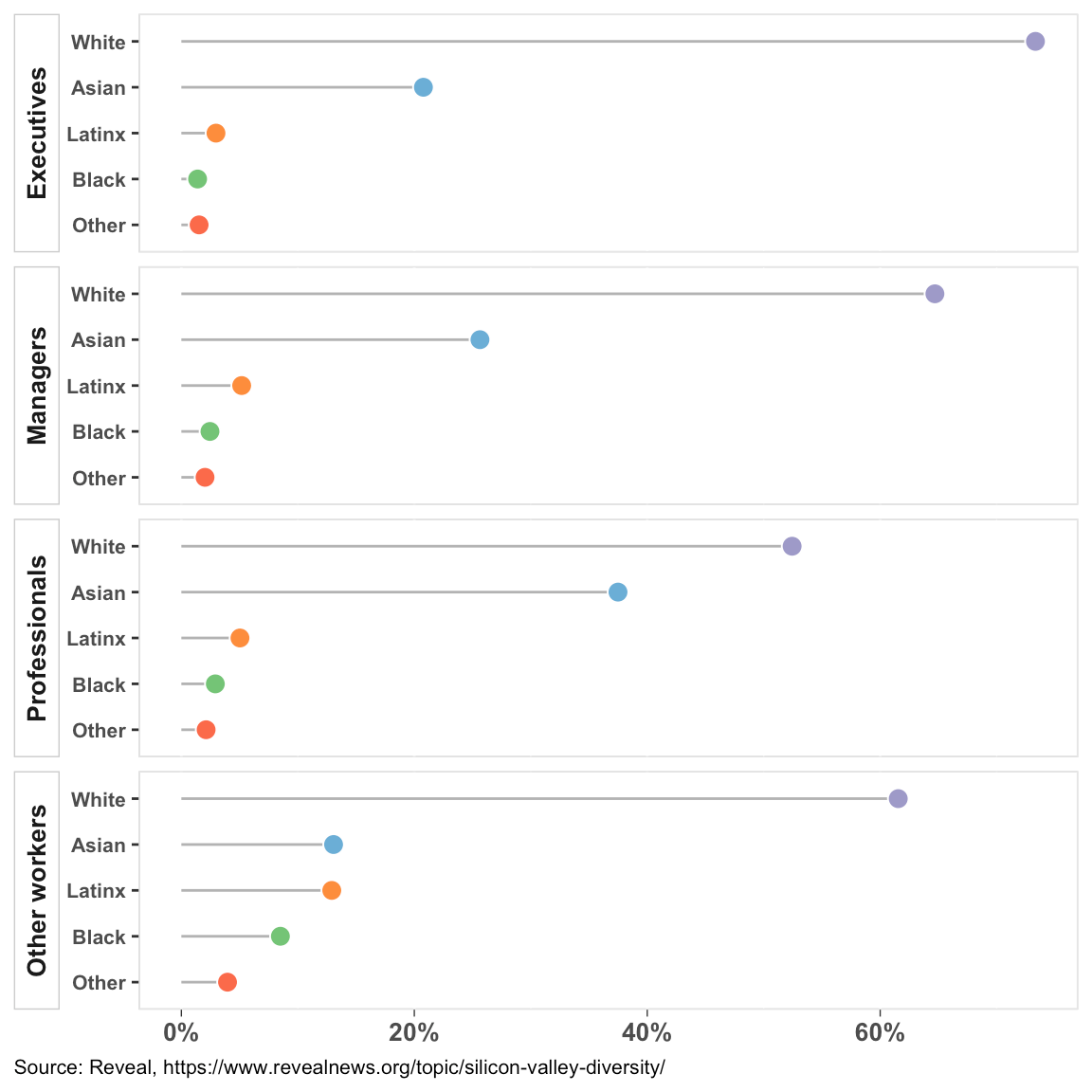Figure 22: A dot chart of race/ethnicity distribution within job category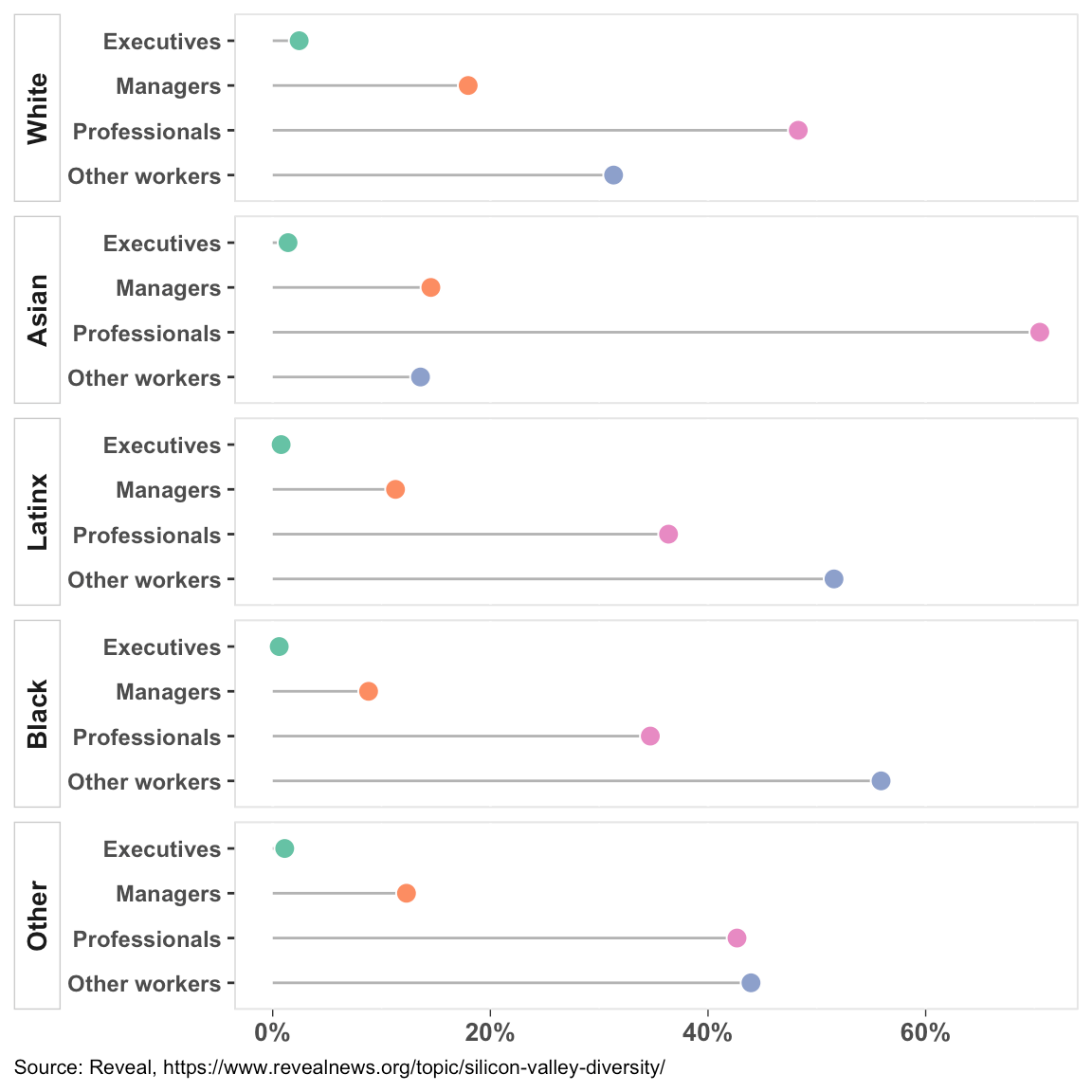Figure 23: A dot chart of job category distribution within race/ethnicity

### What do we see

• In the dot chart of Figure 22, in which we compare ethnicity/race within the job categories, we see:
• since we have more width available compared to the bar charts, the gap between white executives (72%) and Asian executives (21%) looks larger than shown in the bar charts
• The comparison among groups is easier within the panel and with other panels. You don’t need to read the y-axis labels every time.
• You can see the wide gap between white/Asian “professionals” and Latinx/Black/Other “professionals.”
• In the dot chart of Figure 23, in which we compare job categories within the ethnicities/races, we see:
• there are many more Asian “professionals” than there are executives or managers
• there are many more Black/Latinx “other workers” than there are “professionals,” executives, or managers

The dot charts offer more information in a small space compared to the bar or pie charts. The comparison is easier though some precision is lost. You don’t have to use color to distinguish categories similar to bar charts. They also look very clean.

What if we want to compare genders within the job categories and ethnicities/races?

I doubt we will get good, easily explainable graphs. Simple bar charts and pie charts are out. We can try dot charts with two dots for the genders in the data, and side-by-side bar charts.

Here are three attempts:

• Figure 24 shows gender representation within a job category by race. When you add the values of all purple bars or green bars in a job category, you will get a total of 100%.
• Figure 25 is same as Figure 24, but in a dot chart form.
• Figure 26 shows the distribution of all genders and ethnicities/races within a job category. When you add all the values within a job category, you will get a total of 100%.

While Figure 24 and 25 show genders compare among ethnicities/races within a job category, Figure 26 shows how genders from all ethnicities/races compare with each other within a job category.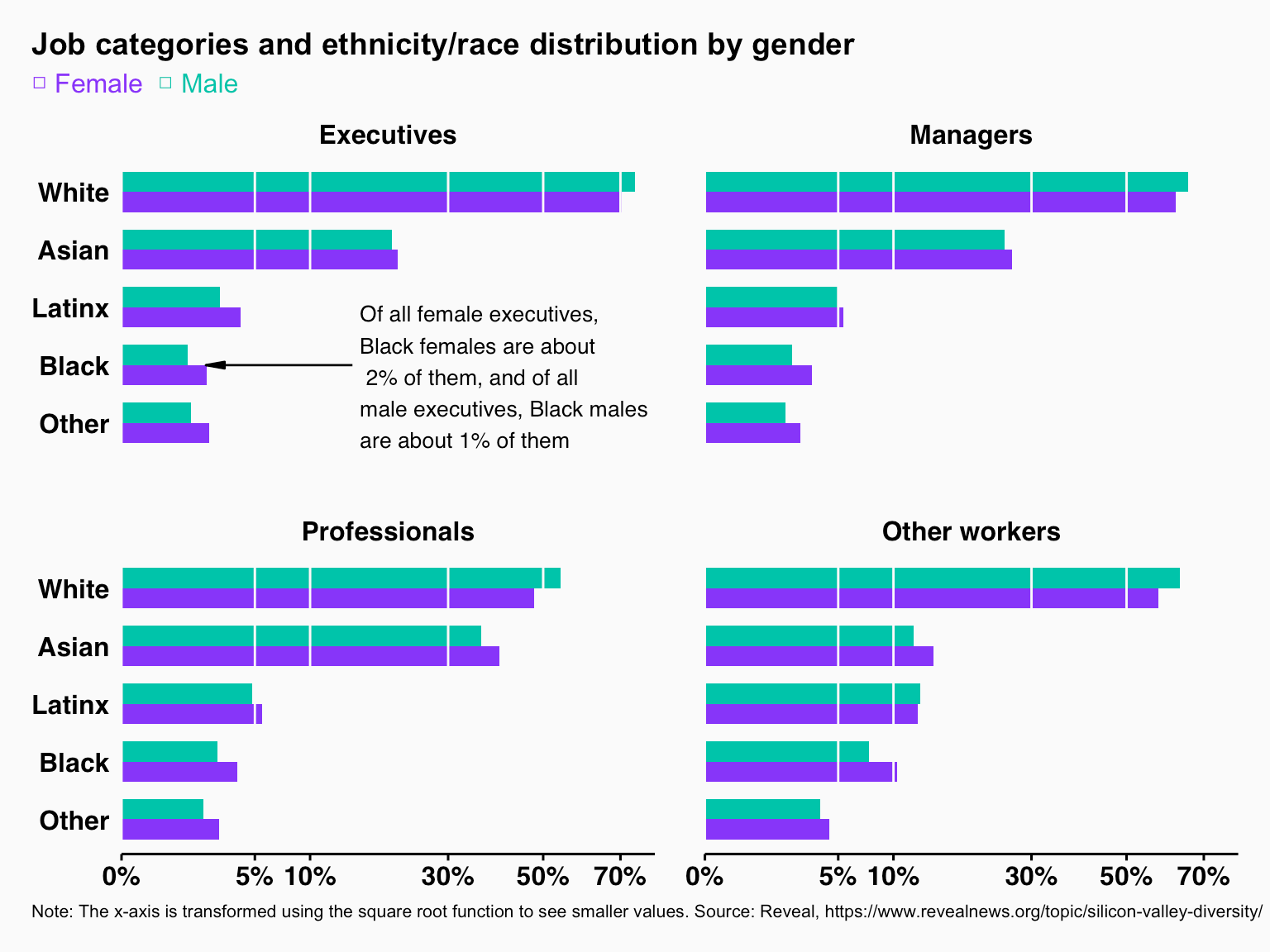Figure 24: Side-by-side bar charts of job categories and ethnicity/race distribution by gender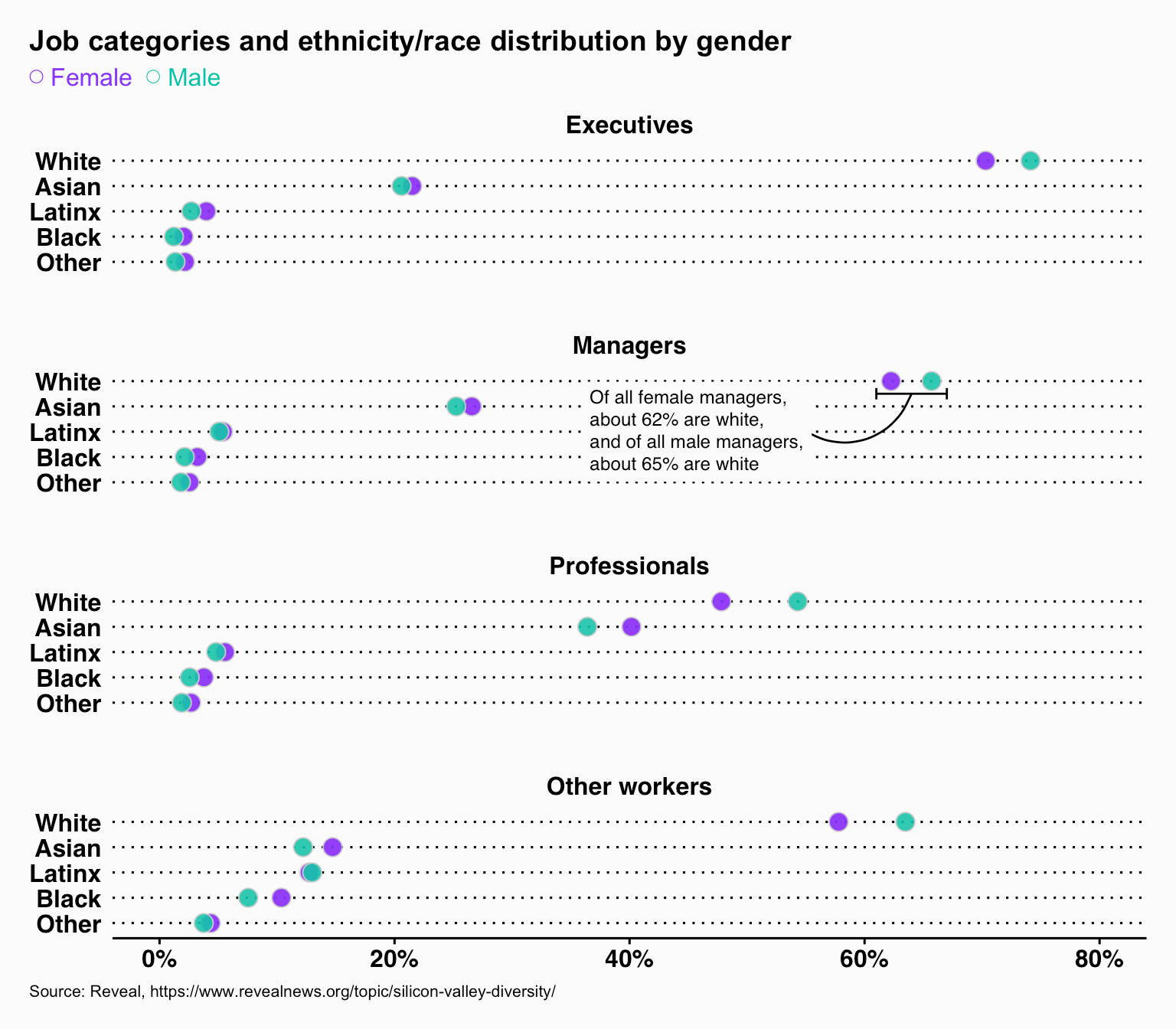Figure 25: Dot charts of job categories and ethnicity/race distribution by gender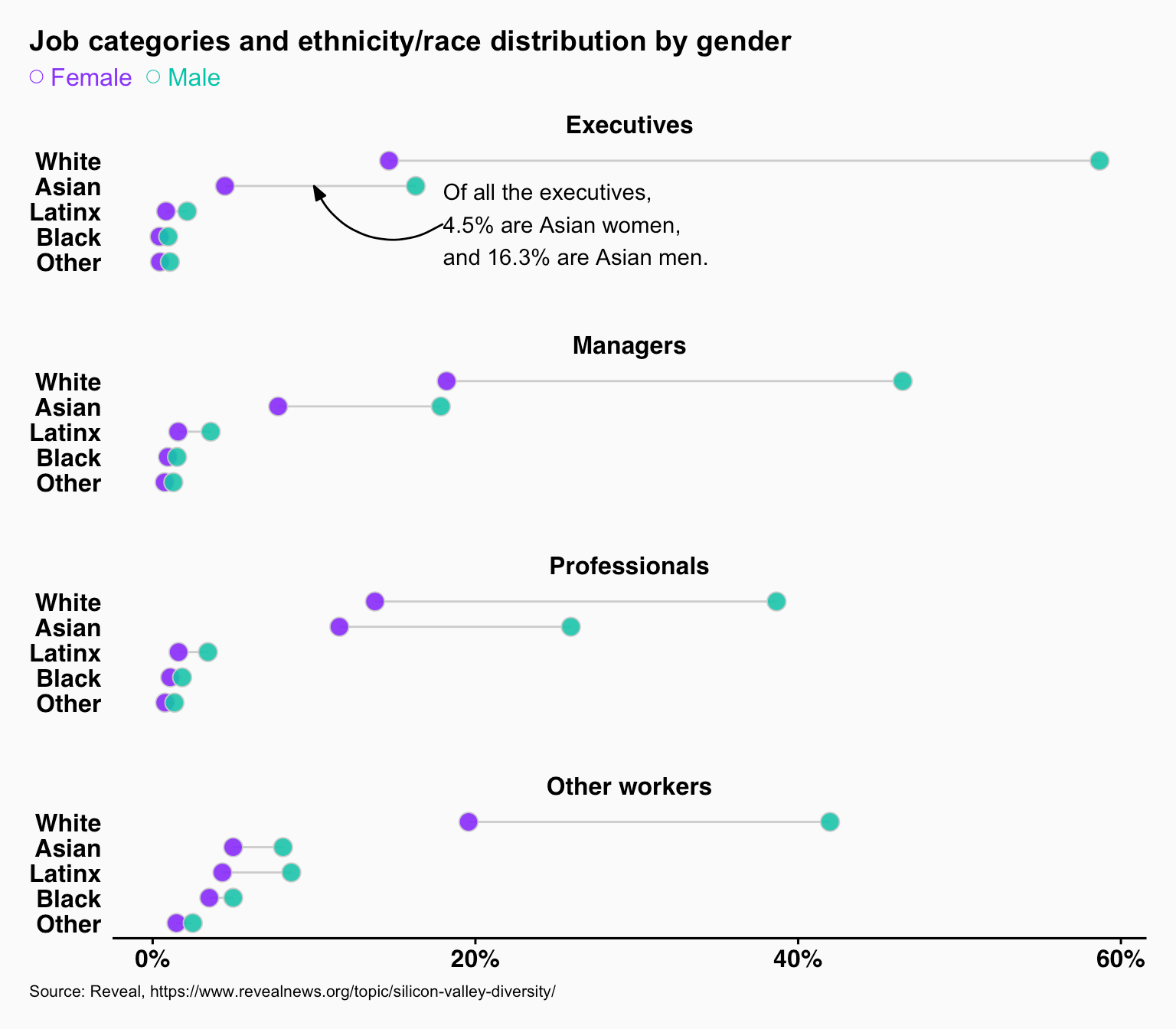Figure 26: Dot charts of job categories and ethnicity/race distribution by gender

## A note on dot charts

As we saw in the previous examples, pie charts aren’t suitable for multi-level comparison, and although bar charts are good alternatives, dot charts offer more flexibility conveying similar information without a loss of perception or understanding. Dot charts don’t require color-coding also because we can use different symbols (patterned bar charts look ugly. I know because I have created many of them before).

## Conclusion

I was surprised by some of the graphs. Pie charts were better in some instances, and side-by-side bar charts were better than dot charts in at least one case. That is why you need to create multiple designs before settling on one. And of course, it also depends on the objective of your overall narrative. For example, Figure 24 and 26 have similar data, but they show two different things. Context is critical. Also, important are design skills – some graphs out of the box may not be ready for sharing, but with editing and annotating, charts can speak for themselves.

## Appendix: R Code

```.wp-block-code {
border: 0;
}

.wp-block-code > div {
overflow: auto;
}

.shcb-language {
border: 0;
clip: rect(1px, 1px, 1px, 1px);
-webkit-clip-path: inset(50%);
clip-path: inset(50%);
height: 1px;
margin: -1px;
overflow: hidden;
position: absolute;
width: 1px;
word-wrap: normal;
word-break: normal;
}

.hljs {
box-sizing: border-box;
}

.hljs.shcb-code-table {
display: table;
width: 100%;
}

.hljs.shcb-code-table > .shcb-loc {
color: inherit;
display: table-row;
width: 100%;
}

.hljs.shcb-code-table .shcb-loc > span {
display: table-cell;
}

.wp-block-code code.hljs:not(.shcb-wrap-lines) {
white-space: pre;
}

.wp-block-code code.hljs.shcb-wrap-lines {
white-space: pre-wrap;
}

.hljs.shcb-line-numbers {
border-spacing: 0;
counter-reset: line;
}

.hljs.shcb-line-numbers > .shcb-loc {
counter-increment: line;
}

.hljs.shcb-line-numbers .shcb-loc > span {
}

.hljs.shcb-line-numbers .shcb-loc::before {
border-right: 1px solid #ddd;
content: counter(line);
display: table-cell;
text-align: right;
-webkit-user-select: none;
-moz-user-select: none;
-ms-user-select: none;
user-select: none;
white-space: nowrap;
width: 1%;
}
```####################################################################################################
## ----setup

knitr::opts_chunk\$set(
echo = FALSE, message = FALSE, warning = FALSE,
fig.width = 6,
fig.align = "center",
dpi = 96
)

library(scales)
library(ggplot2)
library(ggforce)
library(patchwork)
library(dplyr)
library(ggthemes)
library(waffle)
library(RColorBrewer)
library(ggtext)

race_colors <- c("White" = "#9e9ac8", "Asian" = "#6baed6", "Latinx" = "#fd8d3c", "Black" = "#74c476", "Other" = "#fb6a4a")
job_cat_colors <- c("Other workers" = "#8da0cb", "Professionals" = "#e78ac3", "Managers" = "#fc8d62", "Executives" = "#66c2a5")

ordered_lvls_race <- c("White", "Asian", "Latinx", "Black", "Other")
ordered_lvls_job_cat <- c("Executives", "Managers", "Professionals", "Other workers")
####################################################################################################

####################################################################################################
## Simple pie chart in R
par(
mar = c(rep(.8, 4)),
mai = rep(0.1, 4)
)
pie(c(0.3, 0.4, 0.3), labels = c("A", "B", "C"), col = "grey80", border = "white")
####################################################################################################

####################################################################################################
## Three different pie charts with colors and legends
data <- data.frame(
val = c(0.3, 0.4, 0.1, 0.1, 0.05, 0.02, 0.01, 0.02),
cat = LETTERS[1:8],
long_cat = c("Cream of Wheat", "Malt-O-Meal", "Maypo", "Quaker Oats", "Cinnamon Crunch", "Scott's Porage Oats", "Cap'n Crunch", "Cheerios"),
stringsAsFactors = FALSE
)

# copied from Claus Wilke beautiful pie chart in r
# https://github.com/clauswilke/dataviz/blob/master/nested_proportions.Rmd
# I haven't paid attention to know how this works
# create a pie chart from data frame in r

data <- data %>%
arrange(val) %>%
mutate(
end_angle = 2 * pi * cumsum(val) / sum(val),
start_angle = lag(end_angle, default = 0),
mid_angle = 0.5 * (start_angle + end_angle),
hjust = ifelse(mid_angle > pi, 1, 0),
vjust = ifelse(mid_angle < pi / 2 | mid_angle > 3 * pi / 2, 0, 1)
)

rpie <- 1
rlabel_out <- 1.05 * rpie
rlabel_in <- 0.6 * rpie

p1 <- ggplot(data) +
geom_arc_bar(
aes(
x0 = 0, y0 = 0, r0 = 0, r = rpie,
start = start_angle, end = end_angle
),
fill = "grey90",
color = "white"
) +
coord_fixed() +
geom_text(
aes(
x = rlabel_out * sin(mid_angle),
y = rlabel_out * cos(mid_angle),
label = cat,
hjust = hjust, vjust = vjust
),
size = 10 / .pt
) +
theme_void() +
ggtitle("I") +
scale_x_continuous(
name = NULL,
limits = c(-1.5, 1.4),
expand = c(0, 0)
) +
scale_y_continuous(
name = NULL,
limits = c(-1.2, 1.3),
expand = c(0, 0)
) +
theme(plot.title = element_text(color = "#1A3BA5", hjust = 0.5, face = "bold", size = rel(1.5)))

p2 <- ggplot(data) +
geom_arc_bar(
aes(
x0 = 0, y0 = 0, r0 = 0, r = rpie,
start = start_angle, end = end_angle,
fill = cat,
),
color = "white"
) +
coord_fixed() +
geom_text(
aes(
x = rlabel_out * sin(mid_angle),
y = rlabel_out * cos(mid_angle),
label = cat,
hjust = hjust, vjust = vjust
),
size = 10 / .pt
) +
theme_void() +
ggtitle("II") +
scale_x_continuous(
name = NULL,
limits = c(-1.5, 1.4),
expand = c(0, 0)
) +
scale_y_continuous(
name = NULL,
limits = c(-1.2, 1.3),
expand = c(0, 0)
) +
scale_fill_brewer(type = "qual", palette = "Set2") +
theme(legend.position = "none") +
theme(plot.title = element_text(color = "#1A3BA5", hjust = 0.5, face = "bold", size = rel(1.5)))

p3 <- ggplot(data) +
geom_arc_bar(
aes(
x0 = 0, y0 = 0, r0 = 0, r = rpie,
start = start_angle, end = end_angle,
fill = cat,
),
color = "gray85"
) +
coord_fixed() +
geom_text(
aes(
x = rlabel_out * sin(mid_angle),
y = rlabel_out * cos(mid_angle),
label = cat,
hjust = hjust, vjust = vjust
),
size = 10 / .pt
) +
theme_void() +
ggtitle("III") +
scale_x_continuous(
name = NULL,
limits = c(-1.5, 1.4),
expand = c(0, 0)
) +
scale_y_continuous(
name = NULL,
limits = c(-1.2, 1.3),
expand = c(0, 0)
) +
scale_fill_brewer(type = "seq", palette = "Oranges") +
theme(plot.title = element_text(color = "#1A3BA5", hjust = 0.5, face = "bold", size = rel(1.5)))

p1 + p2 + p3
####################################################################################################

####################################################################################################
## Pie charts with data labels
p4 <- ggplot(data) +
geom_arc_bar(
aes(
x0 = 0, y0 = 0, r0 = 0, r = rpie,
start = start_angle, end = end_angle
),
fill = "grey90",
color = "white"
) +
coord_fixed(clip = "off") +
geom_text(
aes(
x = rlabel_out * sin(mid_angle),
y = rlabel_out * cos(mid_angle),
label = paste(cat, percent(val), sep = ": "),
hjust = hjust, vjust = vjust
),
size = 10 / .pt
) +
theme_void() +
ggtitle("Label outside") +
scale_x_continuous(
name = NULL,
limits = c(-1.5, 1.4),
expand = c(0, 0)
) +
scale_y_continuous(
name = NULL,
limits = c(-1.2, 1.3),
expand = c(0, 0)
) +
theme(plot.title = element_text(color = "#1A3BA5", hjust = 0.5, face = "bold", size = rel(1.5)))

p5 <- ggplot(data) +
geom_arc_bar(
aes(
x0 = 0, y0 = 0, r0 = 0, r = rpie,
start = start_angle, end = end_angle
),
fill = "grey90",
color = "white"
) +
coord_fixed(clip = "off") +
geom_text(
aes(
x = rlabel_in * sin(mid_angle),
y = rlabel_in * cos(mid_angle),
label = ifelse(val < .1, NA, paste(cat, percent(val), sep = ": "))
),
size = 10 / .pt
) +
theme_void() +
ggtitle("Label inside") +
scale_x_continuous(
name = NULL,
limits = c(-1.5, 1.4),
expand = c(0, 0)
) +
scale_y_continuous(
name = NULL,
limits = c(-1.2, 1.3),
expand = c(0, 0)
) +
theme(plot.title = element_text(color = "#1A3BA5", hjust = 0.5, face = "bold", size = rel(1.5)))

wrap_plots(p4, p5, heights = 1)
####################################################################################################

####################################################################################################
## Pie chart with collapsed categories

collapsed_data <- data.frame(
val = c(0.3, 0.4, 0.1, 0.2),
cat = c(LETTERS[1:3], "Other"),
stringsAsFactors = FALSE
)

# copied from Claus Wilke https://github.com/clauswilke/dataviz/blob/master/nested_proportions.Rmd
# I haven't paid attention to know how this works

collapsed_data <- collapsed_data %>%
arrange(val) %>%
mutate(
end_angle = 2 * pi * cumsum(val) / sum(val),
start_angle = lag(end_angle, default = 0),
mid_angle = 0.5 * (start_angle + end_angle),
hjust = ifelse(mid_angle > pi, 1, 0),
vjust = ifelse(mid_angle < pi / 2 | mid_angle > 3 * pi / 2, 0, 1)
)

rpie <- 1
rlabel_out <- 1.05 * rpie
rlabel_in <- 0.6 * rpie

p6 <- ggplot(collapsed_data) +
geom_arc_bar(
aes(
x0 = 0, y0 = 0, r0 = 0, r = rpie,
start = start_angle, end = end_angle
),
fill = "grey90",
color = "white"
) +
coord_fixed(clip = "off") +
geom_text(
aes(
x = rlabel_out * sin(mid_angle),
y = rlabel_out * cos(mid_angle),
label = paste(cat, percent(val), sep = ": "),
hjust = hjust, vjust = vjust
),
size = 10 / .pt
) +
theme_void() +
scale_x_continuous(
name = NULL,
limits = c(-1.5, 1.4),
expand = c(0, 0)
) +
scale_y_continuous(
name = NULL,
limits = c(-1.2, 1.3),
expand = c(0, 0)
)

p6
####################################################################################################

####################################################################################################
## Pie chart with a slice highlighted

slice_colors <- rep("grey90", 8)
names(slice_colors) <- LETTERS[1:8]
slice_colors["F"] <- "#F3790C"

p7 <- ggplot(data) +
geom_arc_bar(
aes(
x0 = 0, y0 = 0, r0 = 0, r = rpie,
start = start_angle, end = end_angle,
fill = cat,
),
color = "white"
) +
coord_fixed(clip = "off") +
scale_fill_manual(values = slice_colors) +
geom_text(
aes(
x = rlabel_out * sin(mid_angle),
y = rlabel_out * cos(mid_angle),
label = cat,
hjust = hjust, vjust = vjust
),
size = 10 / .pt
) +
theme_void() +
scale_x_continuous(
name = NULL,
limits = c(-1.5, 1.4),
expand = c(0, 0)
) +
scale_y_continuous(
name = NULL,
limits = c(-1.2, 1.3),
expand = c(0, 0)
) +
theme(legend.position = "none")

p7 <- p7 + geom_curve(aes(x = 0.15, y = 1.14, xend = .6, yend = 1), curvature = -0.6) +
geom_text(aes(x = 0.61, y = 1, label = "25% growth likely\nnext year"),
hjust = 0,
color = "#F3790C"
)

p7
####################################################################################################

####################################################################################################
## Simple bar chart
b1 <- ggplot(data, aes(x = cat, y = val)) +
geom_bar(stat = "identity", fill = "gray80") +
scale_y_continuous(labels = percent) +
theme_wsj() +
theme(
panel.background = element_blank(),
plot.background = element_blank(),
axis.title = element_blank()
)

b1
####################################################################################################

####################################################################################################
## Ordered bar chart
b2 <- ggplot(data, aes(x = reorder(cat, desc(val)), y = val)) +
geom_bar(stat = "identity", fill = "gray80") +
scale_y_continuous(labels = percent) +
theme_wsj() +
theme(
panel.background = element_blank(),
plot.background = element_blank(),
axis.title = element_blank()
)

b2
####################################################################################################

####################################################################################################
## Ordered bar chart with white gridlines
b3 <- ggplot(data, aes(x = reorder(cat, desc(val)), y = val)) +
geom_bar(stat = "identity", fill = "gray80") +
scale_y_continuous(labels = percent) +
theme_wsj() +
geom_hline(yintercept = 1:3 / 10, color = "white") +
theme(
panel.background = element_blank(),
plot.background = element_blank(),
axis.title = element_blank(),
panel.grid.major.y = element_blank()
)

b3
####################################################################################################

####################################################################################################
## Bar charts with long labels
b4 <- ggplot(data, aes(x = reorder(long_cat, desc(val)), y = val)) +
geom_bar(stat = "identity", fill = "gray80") +
scale_y_continuous(labels = percent) +
theme_wsj() +
geom_hline(yintercept = 1:3 / 10, color = "white") +
theme(
panel.background = element_blank(),
plot.background = element_blank(),
axis.title = element_blank(),
panel.grid.major.y = element_blank()
)

b4_i <- b4 + theme(axis.text.x = element_text(angle = 90))

b4_ii <- b4 + theme(axis.text.x = element_text(angle = 30, hjust = 1))

wrap_plots(b4_i, b4_ii, heights = 1)
####################################################################################################

####################################################################################################
## Bar charts with long labels flipped on the y-axis
b5 <- ggplot(data, aes(y = reorder(long_cat, val), x = val)) +
geom_bar(stat = "identity", fill = "gray80") +
scale_x_continuous(labels = percent) +
theme_wsj() +
geom_vline(xintercept = 1:3 / 10, color = "white") +
theme(
panel.background = element_blank(),
plot.background = element_blank(),
axis.title = element_blank(),
panel.grid.major.y = element_blank()
)

b5
####################################################################################################

####################################################################################################
## Bar charts with long labels flipped on the y-axis with some data labels
b6 <- ggplot(data, aes(y = reorder(long_cat, val), x = val)) +
geom_bar(stat = "identity", fill = "gray80") +
scale_x_continuous(labels = percent) +
theme_wsj() +
geom_vline(xintercept = 1:3 / 10, color = "white") +
geom_text(aes(label = ifelse(val < 0.1, percent(val), "")), hjust = -0.1) +
theme(
panel.background = element_blank(),
plot.background = element_blank(),
axis.title = element_blank(),
panel.grid.major.y = element_blank()
)

b6
####################################################################################################

####################################################################################################
## A waffle chart
data2 <- arrange(data, val)
parts_v <- data2\$val * 100
names(parts_v) <- data2\$cat

w1 <- waffle(parts = parts_v, rows = 5, legend_pos = "top", xlab = "1 square equals 1%", reverse = TRUE)

w1 <- w1 + guides(fill = guide_legend(
nrow = 1,
reverse = TRUE,
label.position = "top"
)) +
theme(legend.spacing.x = unit(1.2, "cm"))

w1
####################################################################################################

####################################################################################################
## A simple dot chart
d1 <- ggplot(data, aes(y = reorder(cat, val), x = val)) +
geom_point(shape = 21, fill = "#F3790C", size = 3) +
scale_x_continuous(labels = percent) +
theme_wsj() +
theme(
panel.background = element_blank(),
plot.background = element_blank(),
axis.title = element_blank(),
panel.grid.major.y = element_line(size = 0.4)
)

d1
####################################################################################################

# IT Diversity Silicon Valley  data
# Is Silicon Valley Tech Diversity Possible Now?
# Center for Employment Equity, University of Massachuset
# https://github.com/cirlabs/Silicon-Valley-Diversity-Data
# https://www.umass.edu/employmentequity/sites/default/files/CEE_Diversity%2Bin%2BSilicon%2BValley%2BTech.pdf

####################################################################################################
## Bar chart vs. pie chart comparing job categories of employees in Silicon Valley companies
job_cat_total <- it_data_clean %>%
group_by(job_category) %>%
summarize(total = sum(count))

job_cat_total <- job_cat_total %>%
arrange(total) %>%
mutate(
end_angle = 2 * pi * cumsum(total) / sum(total),
start_angle = lag(end_angle, default = 0),
mid_angle = 0.5 * (start_angle + end_angle),
hjust = ifelse(mid_angle > pi, 1, 0),
vjust = ifelse(mid_angle < pi / 2 | mid_angle > 3 * pi / 2, 0, 1)
) %>%
mutate(pct = total / sum(total))

rpie <- 1
rlabel_out <- 1.05 * rpie
rlabel_in <- 0.6 * rpie

p1_it <- ggplot(job_cat_total) +
geom_arc_bar(
aes(
x0 = 0, y0 = 0, r0 = 0, r = rpie,
start = start_angle, end = end_angle
),
fill = "grey90",
color = "white"
) +
coord_fixed(clip = "off") +
geom_text(
aes(
x = rlabel_out * sin(mid_angle),
y = rlabel_out * cos(mid_angle),
label = job_category,
hjust = hjust, vjust = vjust
),
size = 10 / .pt
) +
theme_void() +
scale_x_continuous(
name = NULL,
limits = c(-1.5, 1.4),
expand = c(0, 0)
) +
scale_y_continuous(
name = NULL,
limits = c(-1.2, 1.3),
expand = c(0, 0)
)

b1_it <- ggplot(job_cat_total, aes(y = reorder(job_category, pct), x = pct)) +
geom_bar(stat = "identity", fill = "gray80") +
scale_x_continuous(labels = percent, limits = c(0, .6)) +
theme_wsj() +
geom_vline(xintercept = 1:6 / 10, color = "white") +
# geom_text(aes(label = ifelse(val < 0.1, percent(val), "")), hjust = -0.1) +
theme(
panel.background = element_blank(),
plot.background = element_blank(),
axis.title = element_blank(),
panel.grid.major.y = element_blank(),
plot.title = element_text(family = "", size = 2)
)

wrap_plots(p1_it, b1_it, heights = 1) +
plot_annotation(
caption = "Source: Reveal, https://www.revealnews.org/topic/silicon-valley-diversity/",
theme = theme(
plot.caption = element_text(family = "", size = 9, hjust = 0),
plot.caption.position = "plot"
)
)
####################################################################################################

####################################################################################################
## Bar chart vs. pie chart comparing ethnicities/races of employees in Silicon Valley companies
race_total <- it_data_clean %>%
group_by(race_short) %>%
summarize(total = sum(count)) %>%
ungroup()

race_total <- race_total %>%
arrange(total) %>%
mutate(
end_angle = 2 * pi * cumsum(total) / sum(total),
start_angle = lag(end_angle, default = 0),
mid_angle = 0.5 * (start_angle + end_angle),
hjust = ifelse(mid_angle > pi, 1, 0),
vjust = ifelse(mid_angle < pi / 2 | mid_angle > 3 * pi / 2, 0, 1)
) %>%
mutate(pct = total / sum(total))

rpie <- 1
rlabel_out <- 1.05 * rpie
rlabel_in <- 0.6 * rpie

p2_it <- ggplot(race_total) +
geom_arc_bar(
aes(
x0 = 0, y0 = 0, r0 = 0, r = rpie,
start = start_angle, end = end_angle
),
fill = "grey90",
color = "white"
) +
coord_fixed(clip = "off") +
geom_text(
aes(
x = rlabel_out * sin(mid_angle),
y = rlabel_out * cos(mid_angle),
label = race_short,
hjust = hjust, vjust = vjust
),
size = 10 / .pt
) +
theme_void() +
scale_x_continuous(
name = NULL,
limits = c(-1.5, 1.4),
expand = c(0, 0)
) +
scale_y_continuous(
name = NULL,
limits = c(-1.2, 1.3),
expand = c(0, 0)
)

b2_it <- ggplot(race_total, aes(y = reorder(race_short, pct), x = pct)) +
geom_bar(stat = "identity", fill = "gray80") +
scale_x_continuous(labels = percent, limits = c(0, .6)) +
theme_wsj() +
geom_vline(xintercept = 1:6 / 10, color = "white") +
# geom_text(aes(label = ifelse(val < 0.1, percent(val), "")), hjust = -0.1) +
theme(
panel.background = element_blank(),
plot.background = element_blank(),
axis.title = element_blank(),
panel.grid.major.y = element_blank(),
plot.title = element_text(family = "", size = 2)
)

wrap_plots(p2_it, b2_it, heights = 1) +
plot_annotation(
caption = "Source: Reveal, https://www.revealnews.org/topic/silicon-valley-diversity/",
theme = theme(
plot.caption = element_text(family = "", size = 9, hjust = 0),
plot.caption.position = "plot"
)
)
####################################################################################################

####################################################################################################
## A pie chart showing ethnicities/races and job categories of employees in Silicon Valley companies
race_job_cat_total <- it_data_clean %>%
group_by(race_short, job_category) %>%
summarize(total = sum(count)) %>%
ungroup() %>%
mutate(race_job_cat = paste(race_short, job_category, sep = "-")) %>%
mutate(pct = total / sum(total))

race_job_cat_total <- race_job_cat_total %>%
arrange(race_short, total) %>%
mutate(
count_total = sum(total),
end_angle = 2 * pi * cumsum(total) / count_total, # ending angle for each pie slice
start_angle = lag(end_angle, default = 0), # starting angle for each pie slice
mid_angle = 0.5 * (start_angle + end_angle), # middle of each pie slice, for the text label
hjust = ifelse(mid_angle > pi, 1, 0),
vjust = ifelse(mid_angle < pi / 2 | mid_angle > 3 * pi / 2, 0, 1),
type = job_category,
label = race_job_cat
)

slice_colors <- c(
brewer.pal(5, "Blues")[-1],
brewer.pal(5, "Greens")[-1],
brewer.pal(5, "Oranges")[-1],
brewer.pal(5, "Reds")[-1],
brewer.pal(5, "Purples")[-1]
)

names(slice_colors) <- race_job_cat_total\$race_job_cat

rpie <- 1
rpie1 <- 0
rpie2 <- 1
rlabel <- 1.02 * rpie

job_cat_race_nested_pie <- ggplot() +
geom_arc_bar(
data = race_job_cat_total,
aes(
x0 = 0, y0 = 0, r0 = rpie1, r = rpie2,
start = start_angle, end = end_angle, fill = race_job_cat
),
color = "white", size = 0.5
) +
geom_text(
data = race_job_cat_total,
aes(
x = rlabel * sin(mid_angle),
y = rlabel * cos(mid_angle),
label = race_job_cat,
hjust = hjust, vjust = vjust
),
size = 12 / .pt
) +
geom_text(
data = race_job_cat_total,
aes(
x = 0.6 * sin(mid_angle),
y = 0.6 * cos(mid_angle),
label = percent(pct, accuracy = 1)
),
size = 10 / .pt,
hjust = 0.5, vjust = 0.5
) +
coord_fixed(clip = "off") +
scale_x_continuous(
limits = c(-1.5, 1.8), expand = c(0, 0), name = "", breaks = NULL, labels = NULL
) +
scale_y_continuous(
limits = c(-1.15, 1.15), expand = c(0, 0), name = "", breaks = NULL, labels = NULL
) +
scale_fill_manual(
values = slice_colors
) +
labs(
caption = "Source: Reveal, https://www.revealnews.org/topic/silicon-valley-diversity/"
) +
theme(
legend.position = "none",
panel.background = element_blank(),
plot.background = element_blank(),
plot.caption = element_text(family = "", size = 8, hjust = 0),
plot.caption.position = "plot",
plot.title.position = "plot",
plot.title = element_text(face = "bold", size = 12)
)

job_cat_race_nested_pie
####################################################################################################

####################################################################################################
## A bar chart showing ethnicities/races and job categories of employees in Silicon Valley companies

ordered_lvls_job_cat_race_combined <- c("White-Executives", "White-Managers", "White-Professionals", "White-Other workers", "Asian-Executives", "Asian-Managers", "Asian-Professionals", "Asian-Other workers", "Latinx-Executives", "Latinx-Managers", "Latinx-Professionals", "Latinx-Other workers", "Black-Executives", "Black-Managers", "Black-Professionals", "Black-Other workers", "Other-Executives", "Other-Managers", "Other-Professionals", "Other-Other workers")

job_cat_race_nested_bar <- ggplot(race_job_cat_total, aes(
y = factor(race_job_cat, levels = rev(ordered_lvls_job_cat_race_combined)),
x = pct,
group = race_short,
fill = job_category
)) +
geom_bar(stat = "identity", position = position_dodge(0.5)) +
scale_fill_brewer(type = "qual", palette = "Set2") +
scale_x_continuous(labels = percent) +
scale_y_discrete(expand = c(0, 0)) +
geom_vline(xintercept = seq(from = 0, to = .3, by = 0.05), color = "white") +
theme_minimal() +
labs(
caption = "Source: Reveal, https://www.revealnews.org/topic/silicon-valley-diversity/"
) +
theme(
legend.position = "top",
legend.title = element_blank(),
panel.grid = element_blank(),
axis.text = element_text(face = "bold", size = 12),
axis.title = element_blank(),
axis.text.y = element_text(hjust = 1),
axis.text.x = element_text(hjust = 0.2),
axis.line = element_line(),
axis.line.y = element_blank(),
axis.ticks.y = element_blank(),
axis.ticks.x = element_line(colour = NULL),
plot.caption = element_text(family = "", size = 8, hjust = 0),
plot.caption.position = "plot",
plot.title.position = "plot",
plot.title = element_text(face = "bold", size = 12)
) +
guides(fill = guide_legend(nrow = 1))

job_cat_race_nested_bar
####################################################################################################

####################################################################################################
## Pie chart panels showing job categories of employees in Silicon Valley companies by ethnicity/race

race_job_cat_total <- it_data_clean %>%
group_by(race_short, job_category) %>%
summarize(total = sum(count)) %>%
mutate(pct = total / sum(total))

race_job_cat_total <- race_job_cat_total %>%
arrange(race_short, total) %>%
group_by(race_short) %>%
mutate(
count_total = sum(total),
end_angle = 2 * pi * cumsum(total) / count_total,
start_angle = lag(end_angle, default = 0),
mid_angle = 0.5 * (start_angle + end_angle),
hjust = ifelse(mid_angle > pi, 1, 0),
vjust = ifelse(mid_angle < pi / 2 | mid_angle > 3 * pi / 2, 0, 1),
type = job_category,
label = job_category
)

race_job_cat_total <- ungroup(race_job_cat_total) %>%
mutate(race_short = factor(race_short, ordered_lvls_race))

rpie <- 1
rpie1 <- 0
rpie2 <- 1
rlabel <- 1.02 * rpie

job_cat_race_panel_pie_v1 <- ggplot() +
geom_arc_bar(
data = race_job_cat_total,
aes(
x0 = 0, y0 = 0, r0 = rpie1, r = rpie2,
start = start_angle, end = end_angle, fill = job_category
),
color = "white", size = 0.5
) +
facet_wrap(~race_short) +
geom_text(
data = race_job_cat_total,
aes(
x = rlabel * sin(mid_angle),
y = rlabel * cos(mid_angle),
label = ifelse(job_category == "Other workers", "Other\nworkers", job_category),
hjust = hjust, vjust = vjust
),
# family = dviz_font_family,
size = 10 / .pt
) +
geom_text(
data = race_job_cat_total,
aes(
x = 0.6 * sin(mid_angle),
y = 0.6 * cos(mid_angle),
label = percent(pct, accuracy = 1)
),
size = 10 / .pt,
hjust = 0.5, vjust = 0.5
) +
coord_fixed(clip = "off") +
scale_x_continuous(
limits = c(-1.5, 1.8), expand = c(0, 0), name = "", breaks = NULL, labels = NULL
) +
scale_y_continuous(
limits = c(-1.15, 1.15), expand = c(0, 0), name = "", breaks = NULL, labels = NULL
) +
scale_fill_brewer(type = "qual", palette = "Set2") +
theme(
legend.position = "none",
panel.background = element_blank(),
plot.background = element_blank()
) +
labs(caption = "Source: Reveal, https://www.revealnews.org/topic/silicon-valley-diversity/")

job_cat_race_panel_pie_v1 <- job_cat_race_panel_pie_v1 +
theme(
strip.text = element_text(face = "bold", size = 12),
strip.background = element_rect(fill = "grey95"),
panel.spacing = unit(1.5, "cm"),
plot.caption = element_text(family = "", size = 8, hjust = 0),
plot.caption.position = "plot"
)

job_cat_race_panel_pie_v1
####################################################################################################

####################################################################################################
## Bar chart panels showing job categories of employees in Silicon Valley companies by ethnicity/race

race_job_cat_total <- it_data_clean %>%
group_by(race_short, job_category) %>%
summarize(total = sum(count)) %>%
mutate(pct = total / sum(total))

race_job_cat_total <- ungroup(race_job_cat_total) %>%
mutate(
race_short = factor(race_short, ordered_lvls_race),
job_category = factor(job_category, rev(ordered_lvls_job_cat))
)

job_cat_race_panel_bar_v1 <- ggplot(race_job_cat_total, aes(
y = job_category,
x = pct,
fill = job_category
)) +
geom_bar(stat = "identity", position = position_dodge(0.5), width = 0.7) +
facet_wrap(~race_short) +
scale_fill_brewer(type = "qual", palette = "Set2") +
scale_x_continuous(labels = percent) +
geom_vline(xintercept = seq(from = 0, to = .6, by = 0.2), color = "white") +
theme_minimal() +
labs(caption = "Source: Reveal, https://www.revealnews.org/topic/silicon-valley-diversity/") +
theme(
legend.position = "none",
legend.title = element_blank(),
panel.grid = element_blank(),
axis.text = element_text(face = "bold", size = 12),
axis.title = element_blank(),
axis.text.y = element_text(hjust = 1),
axis.text.x = element_text(hjust = 0.2),
axis.line = element_line(),
axis.line.y = element_blank(),
axis.ticks.y = element_blank(),
axis.ticks.x = element_line(colour = NULL)
) +
guides(fill = guide_legend(nrow = 1)) +
theme(
strip.text = element_text(face = "bold", size = 12),
strip.background = element_rect(fill = "grey95", color = NA),
panel.spacing = unit(1, "cm"),
plot.caption = element_text(family = "", size = 8, hjust = 0),
plot.caption.position = "plot"
)

job_cat_race_panel_bar_v1
####################################################################################################

####################################################################################################
## Pie chart panels showing ethnicity/race of employees in Silicon Valley companies by job category
race_job_cat_total <- it_data_clean %>%
group_by(job_category, race_short) %>%
summarize(total = sum(count)) %>%
mutate(pct = total / sum(total))

race_job_cat_total <- race_job_cat_total %>%
arrange(job_category, total) %>%
group_by(job_category) %>%
mutate(
count_total = sum(total),
end_angle = 2 * pi * cumsum(total) / count_total, # ending angle for each pie slice
start_angle = lag(end_angle, default = 0), # starting angle for each pie slice
mid_angle = 0.5 * (start_angle + end_angle), # middle of each pie slice, for the text label
hjust = ifelse(mid_angle > pi, 1, 0),
vjust = ifelse(mid_angle < pi / 2 | mid_angle > 3 * pi / 2, 0, 1),
type = job_category,
label = job_category
)

race_job_cat_total <- ungroup(race_job_cat_total) %>%
mutate(
race_short = factor(race_short, rev(ordered_lvls_race)),
job_category = factor(job_category, ordered_lvls_job_cat)
)

rpie <- 1
rpie1 <- 0
rpie2 <- 1
rlabel <- 1.02 * rpie

job_cat_race_panel_pie_v2 <- ggplot() +
geom_arc_bar(
data = race_job_cat_total,
aes(
x0 = 0, y0 = 0, r0 = rpie1, r = rpie2,
start = start_angle, end = end_angle, fill = race_short
),
color = "white", size = 0.5
) +
facet_wrap(~job_category) +
geom_text(
data = race_job_cat_total,
aes(
x = rlabel * sin(mid_angle),
y = rlabel * cos(mid_angle),
label = race_short,
hjust = hjust, vjust = vjust
),
size = 10 / .pt
) +
geom_text(
data = race_job_cat_total,
aes(
x = 0.6 * sin(mid_angle),
y = 0.6 * cos(mid_angle),
label = percent(pct, accuracy = 1)
),
size = 10 / .pt,
hjust = 0.5, vjust = 0.5
) +
coord_fixed(clip = "off") +
scale_x_continuous(
limits = c(-1.5, 1.8), expand = c(0, 0), name = "", breaks = NULL, labels = NULL
) +
scale_y_continuous(
limits = c(-1.15, 1.15), expand = c(0, 0), name = "", breaks = NULL, labels = NULL
) +
labs(caption = "Source: Reveal, https://www.revealnews.org/topic/silicon-valley-diversity/") +
scale_fill_manual(values = race_colors) +
theme(
legend.position = "none",
panel.background = element_blank(),
plot.background = element_blank()
)

job_cat_race_panel_pie_v2 <- job_cat_race_panel_pie_v2 +
theme(
strip.text = element_text(face = "bold", size = 12),
strip.background = element_rect(fill = "grey95"),
panel.spacing = unit(1.5, "cm"),
plot.caption = element_text(family = "", size = 8, hjust = 0),
plot.caption.position = "plot"
)

job_cat_race_panel_pie_v2
####################################################################################################

####################################################################################################
## Bar chart panels showing ethnicity/race of employees in Silicon Valley companies by job category

race_job_cat_total <- ungroup(race_job_cat_total) %>%
mutate(
race_short = factor(race_short, rev(ordered_lvls_race)),
job_category = factor(job_category, ordered_lvls_job_cat)
)

job_cat_race_panel_bar_v2 <- ggplot(race_job_cat_total, aes(
y = race_short,
x = pct,
fill = race_short
)) +
geom_bar(stat = "identity", position = position_dodge(0.5), width = 0.7) +
facet_wrap(~job_category) +
scale_fill_manual(values = race_colors) +
scale_x_continuous(labels = percent) +
scale_y_discrete(expand = c(0, 0)) +
geom_vline(xintercept = seq(from = 0, to = .6, by = 0.2), color = "white") +
theme_minimal() +
labs(caption = "Source: Reveal, https://www.revealnews.org/topic/silicon-valley-diversity/") +
theme(
legend.position = "none",
legend.title = element_blank(),
panel.grid = element_blank(),
axis.text = element_text(face = "bold", size = 12),
axis.title = element_blank(),
axis.text.y = element_text(hjust = 1),
axis.text.x = element_text(hjust = 0.2),
axis.line = element_line(),
axis.line.y = element_blank(),
axis.ticks.y = element_blank(),
axis.ticks.x = element_line(colour = NULL)
) +
guides(fill = guide_legend(nrow = 1)) +
theme(
strip.text = element_text(face = "bold", size = 12),
strip.background = element_rect(fill = "grey95", color = NA),
panel.spacing = unit(1, "cm"),
plot.caption = element_text(family = "", size = 8, hjust = 0),
plot.caption.position = "plot"
)

job_cat_race_panel_bar_v2
####################################################################################################

####################################################################################################
## A dot chart of race/ethnicity distribution within job category

race_job_cat_distribution_within_jobcat <- it_data_clean %>%
group_by(race_short, job_category) %>%
summarize(total = sum(count)) %>%
group_by(race_short) %>%
mutate(pct = total / sum(total))

race_job_cat_distribution_within_race <- it_data_clean %>%
group_by(race_short, job_category) %>%
summarize(total = sum(count)) %>%
group_by(job_category) %>%
mutate(pct = total / sum(total))

race_job_cat_distribution_within_race <- ungroup(race_job_cat_distribution_within_race) %>%
mutate(
race_short = factor(race_short, rev(ordered_lvls_race)),
job_category = factor(job_category, ordered_lvls_job_cat)
)

race_within_job_cat_dot_plot <- ggplot(
race_job_cat_distribution_within_race,
aes(
x = pct,
y = race_short,
fill = race_short
)
) +
geom_segment(aes(x = 0, y = race_short, xend = pct, yend = race_short), color = "grey70") +
geom_point(shape = 21, color = "white", size = rel(3.5)) +
facet_grid(job_category ~ .,
scales = "free_y",
space = "free",
switch = "y"
) +
scale_fill_manual(values = race_colors) +
labs(caption = "Source: Reveal, https://www.revealnews.org/topic/silicon-valley-diversity/")

race_within_job_cat_dot_plot <- race_within_job_cat_dot_plot +
scale_x_continuous(labels = percent) +
theme(
panel.grid.major.y = element_line(size = rel(0.075), linetype = "dashed"),
strip.background.y = element_rect(fill = "white", color = "grey80"),
axis.ticks.x = element_line(size = rel(0.5)),
strip.text.y = element_text(angle = 180, face = "bold", size = rel(1.15)),
strip.placement = "outside",
axis.text = element_text(face = "bold", size = 10),
panel.background = element_rect(fill = NA, color = "gray80"),
legend.position = "none"
)

race_within_job_cat_dot_plot <- race_within_job_cat_dot_plot + theme(
panel.border = element_rect(color = "grey90", fill = NA),
axis.text.y = element_text(size = rel(0.8)),
axis.title = element_blank(),
plot.caption = element_text(family = "", size = 8, hjust = 0),
plot.caption.position = "plot"
)

race_within_job_cat_dot_plot
####################################################################################################

####################################################################################################
## A dot chart of job category distribution within race/ethnicity

race_job_cat_distribution_within_jobcat <- ungroup(race_job_cat_distribution_within_jobcat) %>%
mutate(
race_short = factor(race_short, ordered_lvls_race),
job_category = factor(job_category, rev(ordered_lvls_job_cat))
)

job_cat_within_race_dot_plot <- ggplot(
race_job_cat_distribution_within_jobcat,
aes(
x = pct,
y = job_category,
fill = job_category
)
) +
geom_segment(aes(x = 0, y = job_category, xend = pct, yend = job_category), color = "grey70") +
geom_point(shape = 21, color = "white", size = rel(3.5)) +
facet_grid(race_short ~ .,
scales = "free_y",
space = "free",
switch = "y"
) +
scale_fill_manual(values = job_cat_colors) +
labs(caption = "Source: Reveal, https://www.revealnews.org/topic/silicon-valley-diversity/")

job_cat_within_race_dot_plot <- job_cat_within_race_dot_plot +
scale_x_continuous(labels = percent) +
theme(
panel.grid.major.y = element_line(size = rel(0.075), linetype = "dashed"),
strip.background.y = element_rect(fill = "white", color = "grey80"),
axis.ticks.x = element_line(size = rel(0.5)),
strip.text.y = element_text(angle = 180, face = "bold", size = rel(1.2)),
strip.placement = "outside",
axis.text = element_text(face = "bold", size = 10),
panel.background = element_rect(fill = NA, color = "gray80"),
legend.position = "none"
)

job_cat_within_race_dot_plot <- job_cat_within_race_dot_plot + theme(
panel.border = element_rect(color = "grey90", fill = NA),
axis.text.y = element_text(size = rel(0.9)),
axis.title = element_blank(),
plot.caption = element_text(family = "", size = 8, hjust = 0),
plot.caption.position = "plot"
)

job_cat_within_race_dot_plot
####################################################################################################

####################################################################################################
## Side-by-side bar charts of job categories and ethnicity/race distribution by gender

#  https://blog.datawrapper.de/gendercolor/
# https://stackoverflow.com/questions/17083362/colorize-parts-of-the-title-in-a-plot
# https://stackoverflow.com/questions/52902946/using-unicode-characters-as-shape

gender_ratio_by_job_cat_role <- it_data_clean %>%
group_by(job_category, race_short, gender) %>%
summarize(total = sum(count)) %>%
group_by(job_category, gender) %>%
mutate(pct = total / sum(total))

gender_ratio_by_job_cat_role <- ungroup(gender_ratio_by_job_cat_role) %>%
mutate(
race_short = factor(race_short, rev(ordered_lvls_race)),
job_category = factor(job_category, ordered_lvls_job_cat)
)

gender_job_cat_race_bar <- ggplot(
gender_ratio_by_job_cat_role,
aes(y = race_short, x = pct, group = gender, fill = gender)
) +
geom_bar(stat = "identity", position = "dodge", width = 0.7) +
scale_fill_manual(values = c("Female" = "#8700f9", "Male" = "#00c4aa")) +
scale_x_sqrt(
labels = scales::percent_format(accuracy = 1),
limits = c(0, .8),
breaks = c(0, 0.05, .1, .3, .5, .7),
expand = c(0, 0)
) +
facet_wrap(job_category ~ .)

gender_job_cat_race_bar <- gender_job_cat_race_bar +
ggtitle(
label = "Job categories and ethnicity/race distribution by gender",
subtitle = paste(
"<b style='color:#8700f9'>\u25A1 Female</b>",
"<b style='color:#00c4aa'>\u25A1 Male</b>"
)
) +
labs(caption = "Note: The x-axis is transformed using the square root function to see smaller values. Source: Reveal, https://www.revealnews.org/topic/silicon-valley-diversity/") +
geom_vline(xintercept = c(0, 0.05, .1, .3, .5, .7), color = "grey98") +
theme_wsj() +
theme(
strip.text.x = element_text(face = "bold", size = 12),
strip.background = element_rect(fill = "grey98"),
panel.background = element_rect(fill = "grey98"),
plot.background = element_rect(fill = "grey98"),
panel.grid.major = element_blank(),
panel.spacing = unit(0.8, "cm"),
legend.position = "none",
legend.title = element_blank(),
plot.title = element_text(size = 14, family = ""),
plot.title.position = "plot",
plot.subtitle = ggtext::element_markdown(
lineheight = 1.1,
family = "Arial Unicode MS",
size = 12
),
plot.caption = element_text(family = "", size = 8, hjust = 0),
plot.caption.position = "plot"
)

annotation_df <- data.frame(
label = "Of all female executives,\nBlack females are about\n 2% of them, and of all\nmale executives, Black males\nare about 1% of them",
x = .16,
y = 1.8,
gender = "Male",
job_category = "Executives",
race_short = "Black"
)

gender_job_cat_race_bar <- gender_job_cat_race_bar +
geom_curve(
data = data.frame(x = .02, y = 2, xend = 0.15, yend = 2, gender = "Male", job_category = "Executives"),
aes(x = x, y = y, xend = xend, yend = yend),
curvature = 0,
arrow = arrow(angle = 10, ends = "first", type = "closed", length = unit(0.12, "inches"))
) +
geom_text(
data = annotation_df,
aes(x = x, y = y, label = label),
size = rel(3.5),
hjust = 0,
family = "sans",
)

gender_job_cat_race_bar
####################################################################################################

####################################################################################################
## Dot charts of job categories and ethnicity/race distribution by gender

gender_ratio_by_job_cat_role <- it_data_clean %>%
group_by(job_category, race_short, gender) %>%
summarize(total = sum(count)) %>%
group_by(job_category, gender) %>%
mutate(pct = total / sum(total))

gender_ratio_by_job_cat_role <- ungroup(gender_ratio_by_job_cat_role) %>%
mutate(
race_short = factor(race_short, rev(ordered_lvls_race)),
job_category = factor(job_category, ordered_lvls_job_cat)
)

gender_job_cat_race_dot <- ggplot(
gender_ratio_by_job_cat_role,
aes(y = race_short, x = pct, group = gender, fill = gender)
) +
geom_point(shape = 21, color = "grey80", size = 4, alpha = 0.9) +
scale_fill_manual(values = c("Female" = "#8700f9", "Male" = "#00c4aa")) +
scale_x_continuous(
labels = scales::percent_format(accuracy = 1),
limits = c(0, .8),
breaks = seq(from = 0, to = 8, by = 2) / 10
) +
facet_wrap(job_category ~ ., ncol = 1)

gender_job_cat_race_dot <- gender_job_cat_race_dot +
ggtitle(
label = "Job categories and ethnicity/race distribution by gender",
subtitle = paste(
"<b style='color:#8700f9'>\u25EF Female</b>",
"<b style='color:#00c4aa'>\u25EF Male</b>"
)
) +
labs(caption = "Source: Reveal, https://www.revealnews.org/topic/silicon-valley-diversity/") +
theme_wsj() +
theme(
strip.text.x = element_text(face = "bold", size = 12),
strip.background = element_rect(fill = "grey98"),
panel.background = element_rect(fill = "grey98"),
plot.background = element_rect(fill = "grey98"),
panel.spacing = unit(0.8, "cm"),
legend.position = "none",
legend.title = element_blank(),
plot.title = element_text(size = 14, family = ""),
plot.title.position = "plot",
plot.subtitle = ggtext::element_markdown(
lineheight = 1.1,
family = "Arial Unicode MS",
size = 12
),
plot.caption = element_text(family = "", size = 8, hjust = 0),
plot.caption.position = "plot"
)

annotation_df <- data.frame(
label = "Of all female managers,<br>about 62% are white,<br> and of all male managers,<br> about 65% are white",
x = .36,
y = 3,
gender = "Male",
job_category = "Managers",
race_short = "Asian"
)

curve_ann_df <- data.frame(x = .55, y = 3, xend = 0.64, yend = 4.5, gender = "Male", job_category = "Managers")

curve_ann_tiny_line_df <- data.frame(xmin = .61, y = 4.5, xmax = 0.67, gender = "Male", job_category = "Managers")

gender_job_cat_race_dot <- gender_job_cat_race_dot +
geom_curve(
data = curve_ann_df,
aes(x = x, y = y, xend = xend, yend = yend),
curvature = .5
) +
geom_errorbar(
data = curve_ann_tiny_line_df,
aes(xmin = xmin, y = y, xmax = xmax),
inherit.aes = F,
width = 0.45
) +
ggtext::geom_richtext(
data = annotation_df,
aes(x = x, y = y, label = label),
size = rel(3.2),
hjust = 0,
family = "sans",
label.color = "grey98",
fill = "grey98"
)

gender_job_cat_race_dot
####################################################################################################

####################################################################################################
## Dot charts of job categories and ethnicity/race distribution by gender
gender_within_job_cat <- it_data_clean %>%
group_by(race_short, job_category, gender) %>%
summarize(total = sum(count)) %>%
group_by(job_category) %>%
mutate(pct = total / sum(total))

gender_within_job_cat <- ungroup(gender_within_job_cat) %>%
mutate(
race_short = factor(race_short, rev(ordered_lvls_race)),
job_category = factor(job_category, ordered_lvls_job_cat)
)

gender_within_job_cat_dot_plt <- ggplot(gender_within_job_cat, aes(y = race_short, x = pct, group = gender, fill = gender)) +
geom_line(aes(group = race_short), color = "grey80") +
geom_point(shape = 21, color = "grey80", size = 4, alpha = 0.9) +
scale_fill_manual(values = c("Female" = "#8700f9", "Male" = "#00c4aa")) +
scale_x_continuous(labels = scales::percent_format()) +
facet_wrap(job_category ~ ., ncol = 1)

gender_within_job_cat_dot_plt <- gender_within_job_cat_dot_plt + ggtitle(
label = "Job categories and ethnicity/race distribution by gender",
subtitle = paste(
"<b style='color:#8700f9'>\u25EF Female</b>",
"<b style='color:#00c4aa'>\u25EF Male</b>"
)
) +
theme_wsj() +
labs(caption = "Source: Reveal, https://www.revealnews.org/topic/silicon-valley-diversity/") +
theme(
panel.background = element_rect(fill = "grey98"),
plot.background = element_rect(fill = "grey98"),
panel.spacing = unit(0.8, "cm"),
legend.position = "none",
legend.title = element_blank(),
plot.title = element_text(size = 14, family = ""),
plot.title.position = "plot",
plot.subtitle = ggtext::element_markdown(
lineheight = 1.1,
family = "Arial Unicode MS",
size = 12
),
panel.grid.major = element_blank(),
plot.caption = element_text(family = "", size = 8, hjust = 0),
plot.caption.position = "plot"
)

gender_within_job_cat_dot_plt <- gender_within_job_cat_dot_plt +
theme(
strip.text.x = element_text(face = "bold", size = 12),
strip.placement = "outside",
strip.background = element_rect(fill = "grey98", color = "grey40")
)

gender_within_job_cat_dot_plt <- gender_within_job_cat_dot_plt +
geom_curve(
data = data.frame(x = .1, y = 4, xend = .18, yend = 2.5, job_category = "Executives", gender = "Male", race_short = "Asian"),
aes(x = x, y = y, xend = xend, yend = yend),
arrow = arrow(angle = 20, ends = "first", type = "closed", length = unit(0.1, "inches"))
) +
geom_text(
data = data.frame(
label = "Of all the executives,\n4.5% are Asian women,\nand 16.3% are Asian men.",
x = .18,
y = 2.5,
gender = "Male",
job_category = "Executives",
race_short = "Asian"
),
aes(x = x, y = y, label = label),
hjust = 0
)

gender_within_job_cat_dot_plt
####################################################################################################
```Code language: R (r)```